# RD Sharma Solutions for Class 9 Maths Chapter 6 Factorization of Polynomials

## RD Sharma Solutions for Class 9 Maths Chapter 6 – Free PDF Download

RD Sharma Solutions for Class 9 Maths Chapter 6 Factorization of Polynomials are provided here to enhance learning skills among students. In this chapter, students will learn various terms such as polynomial, degree of polynomial, factors, multiples and zeros of a polynomial. A polynomial is defined as an expression which is composed of variables, constants and exponents, that are combined using mathematical operations such as addition, subtraction, multiplication and division (No division operation by a variable). Based on the numbers of terms present in the expression, it is classified as monomial, binomial, and trinomial. Students can download RD Sharma Solutions for Class 9 to learn all chapters in depth listed in the textbook.

The PDF of these solutions can be easily downloaded from the links below. BYJU’S subject matter experts have uniquely formulated the solutions for better understanding of covered concepts. By practising RD Sharma Solutions for Class 9, students can fulfil their wish by securing good marks in board exams. RD Sharma Solutions are the best study guide to improve the skills vital from an exam perspective.

## Download PDF of RD Sharma Solutions for Class 9 Maths Chapter 6 Factorization of Polynomials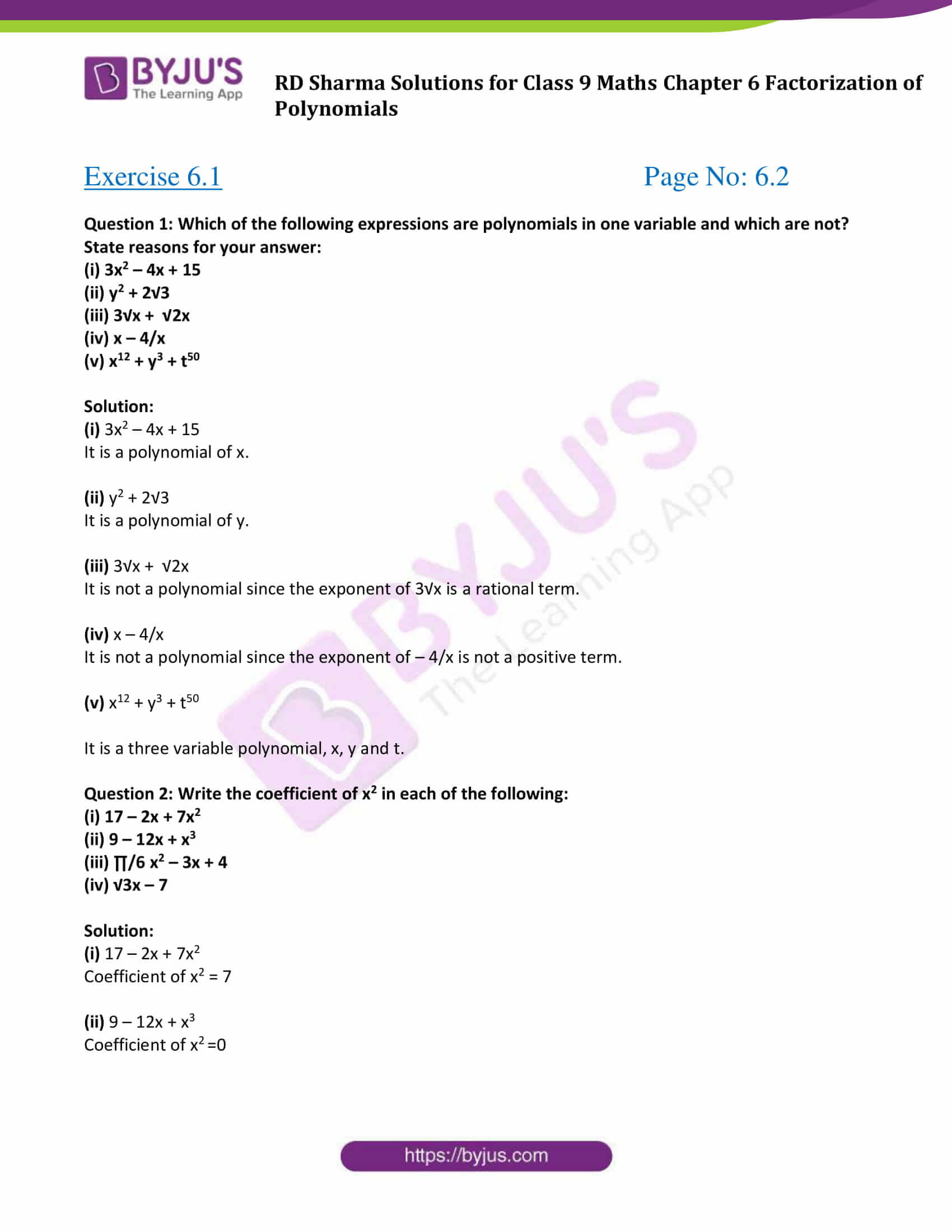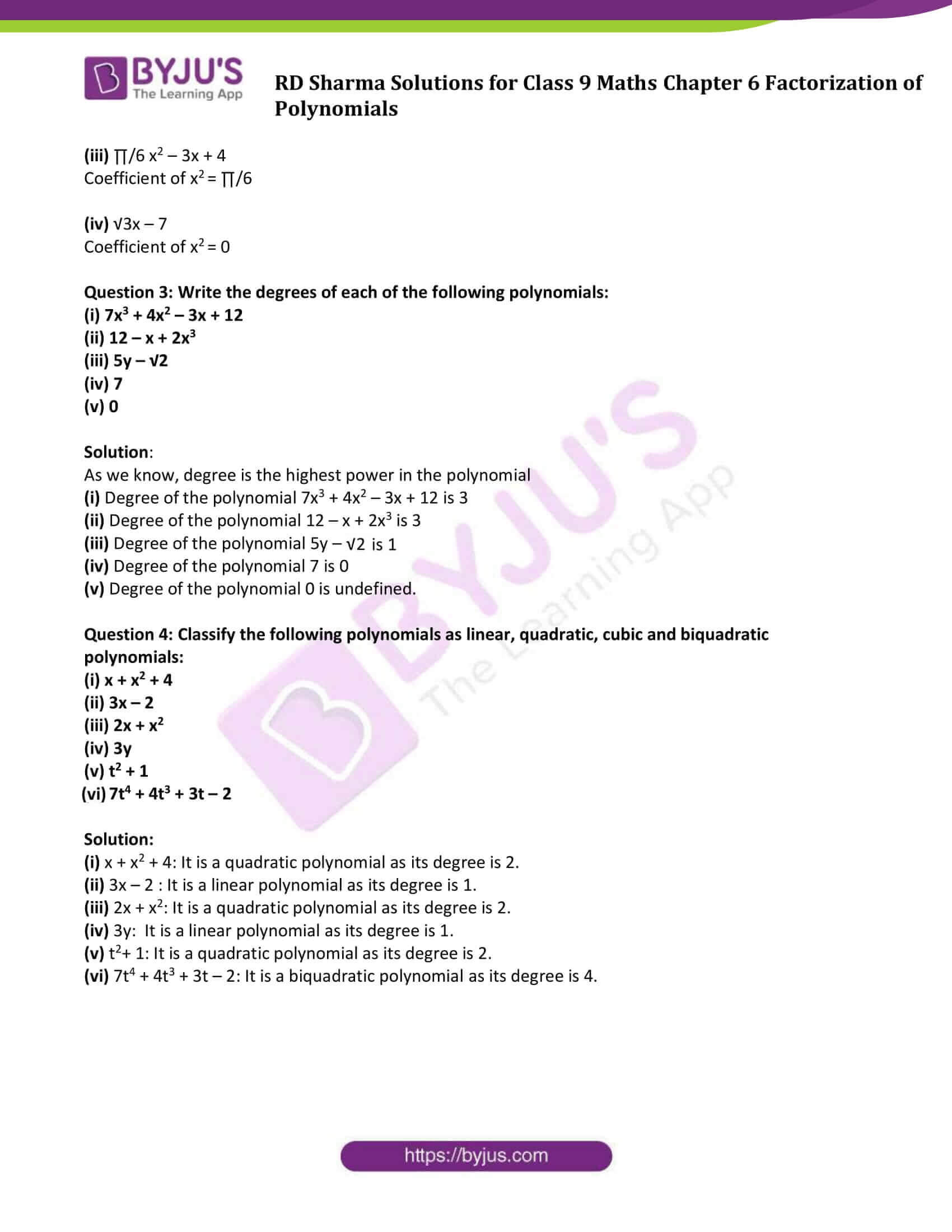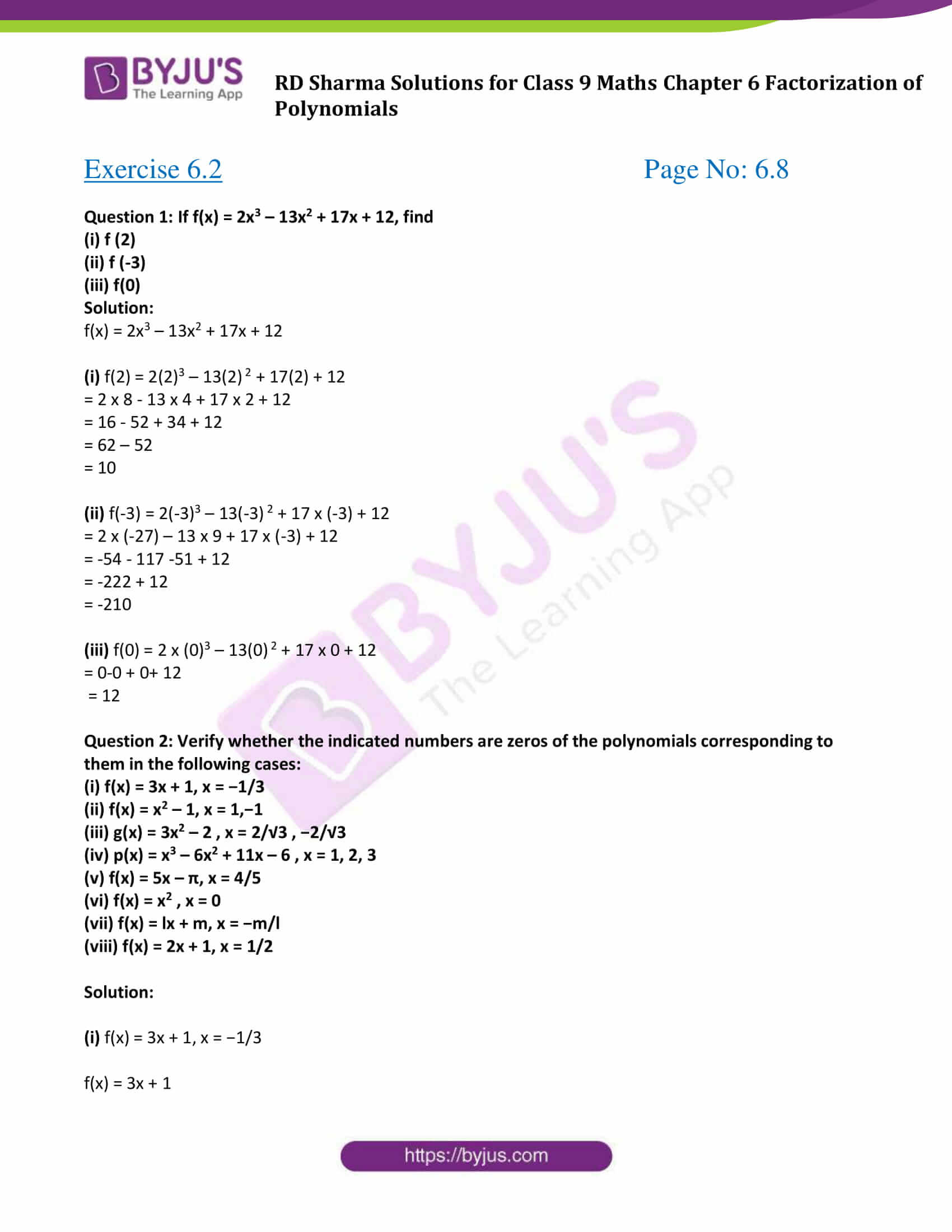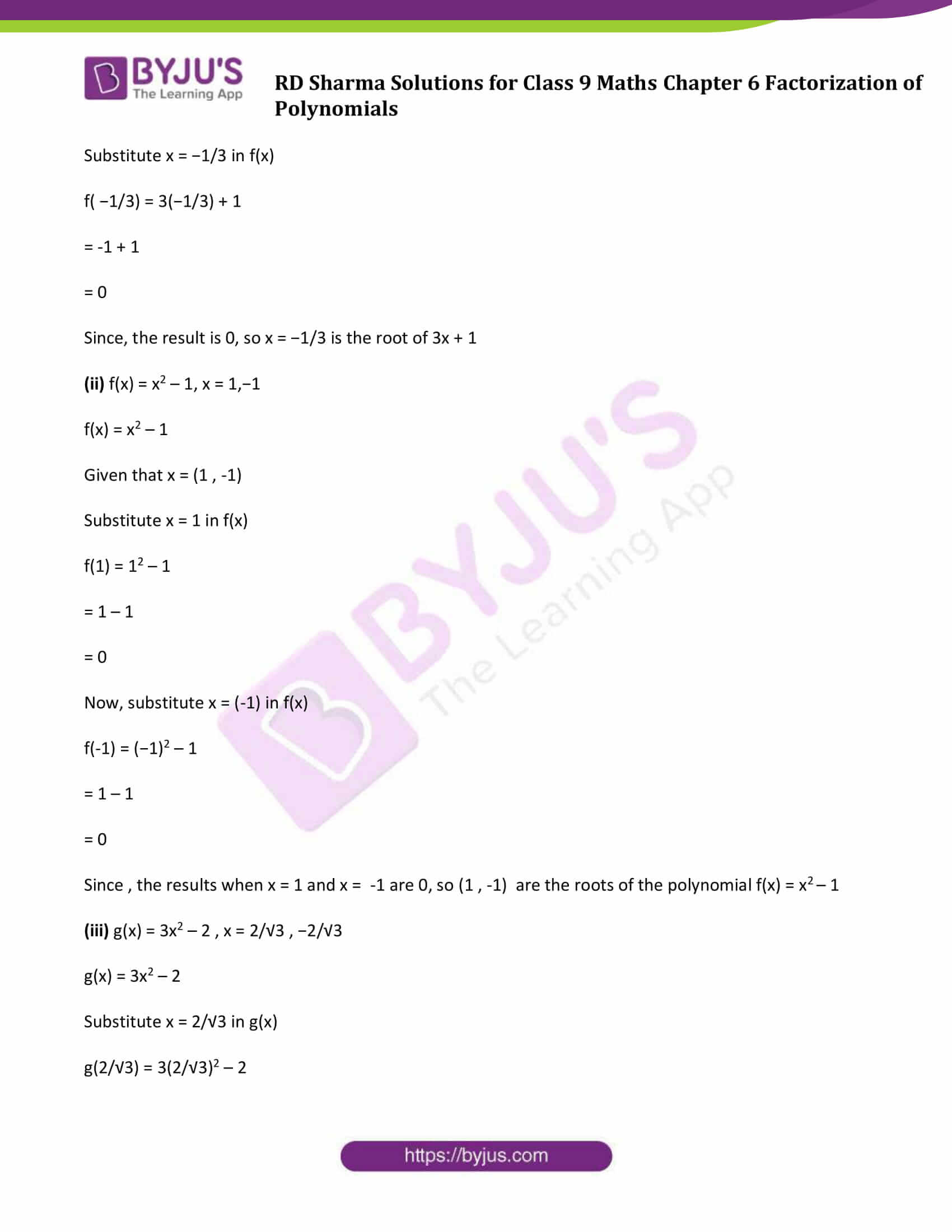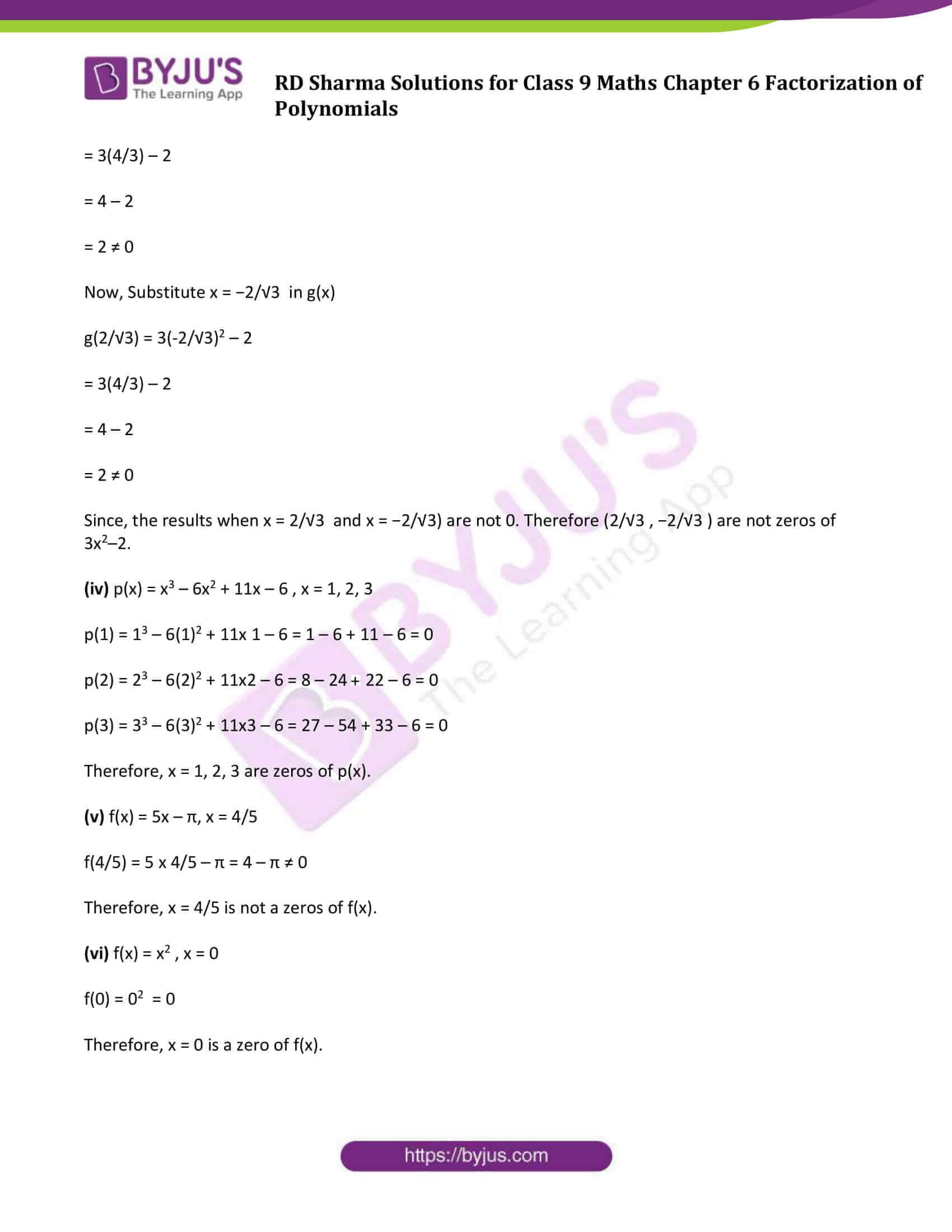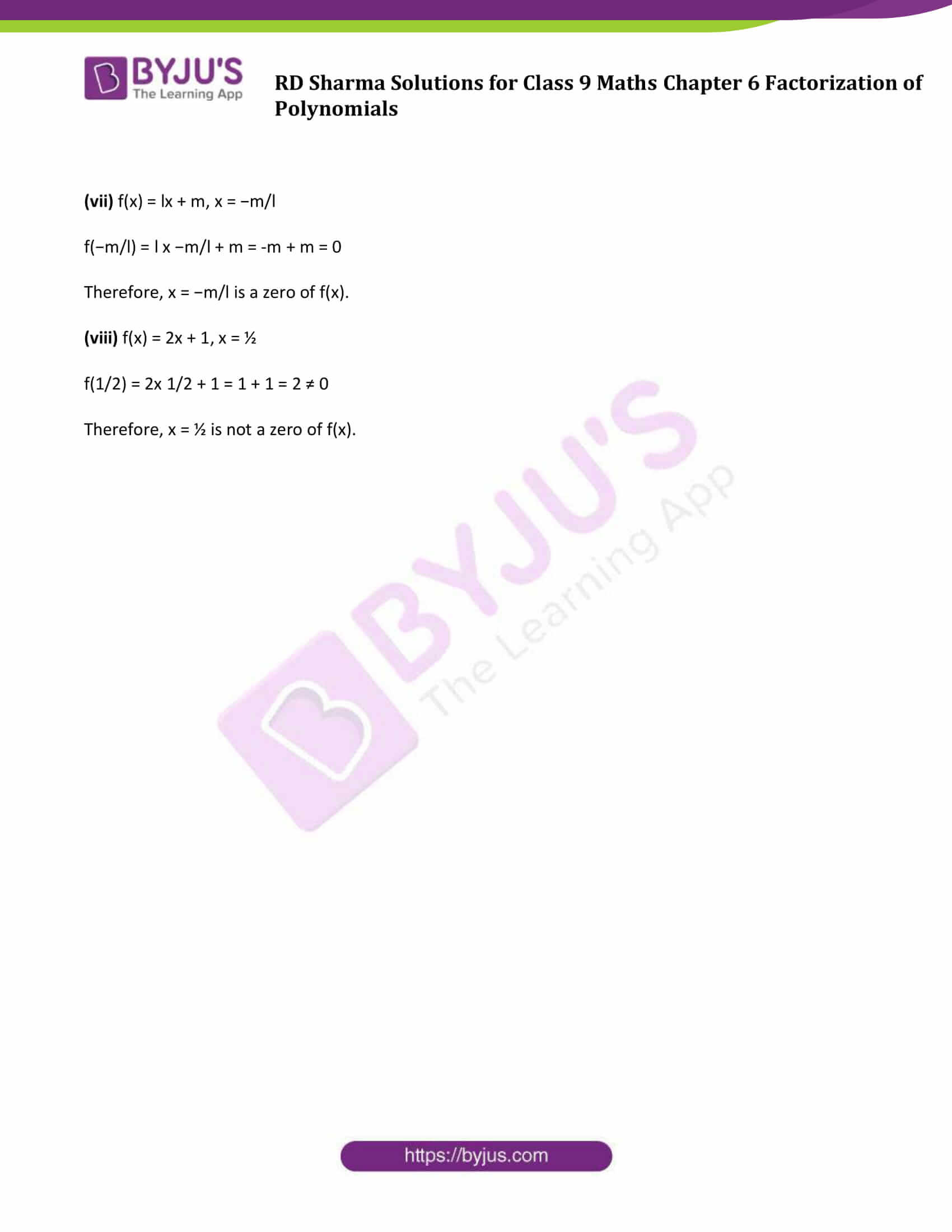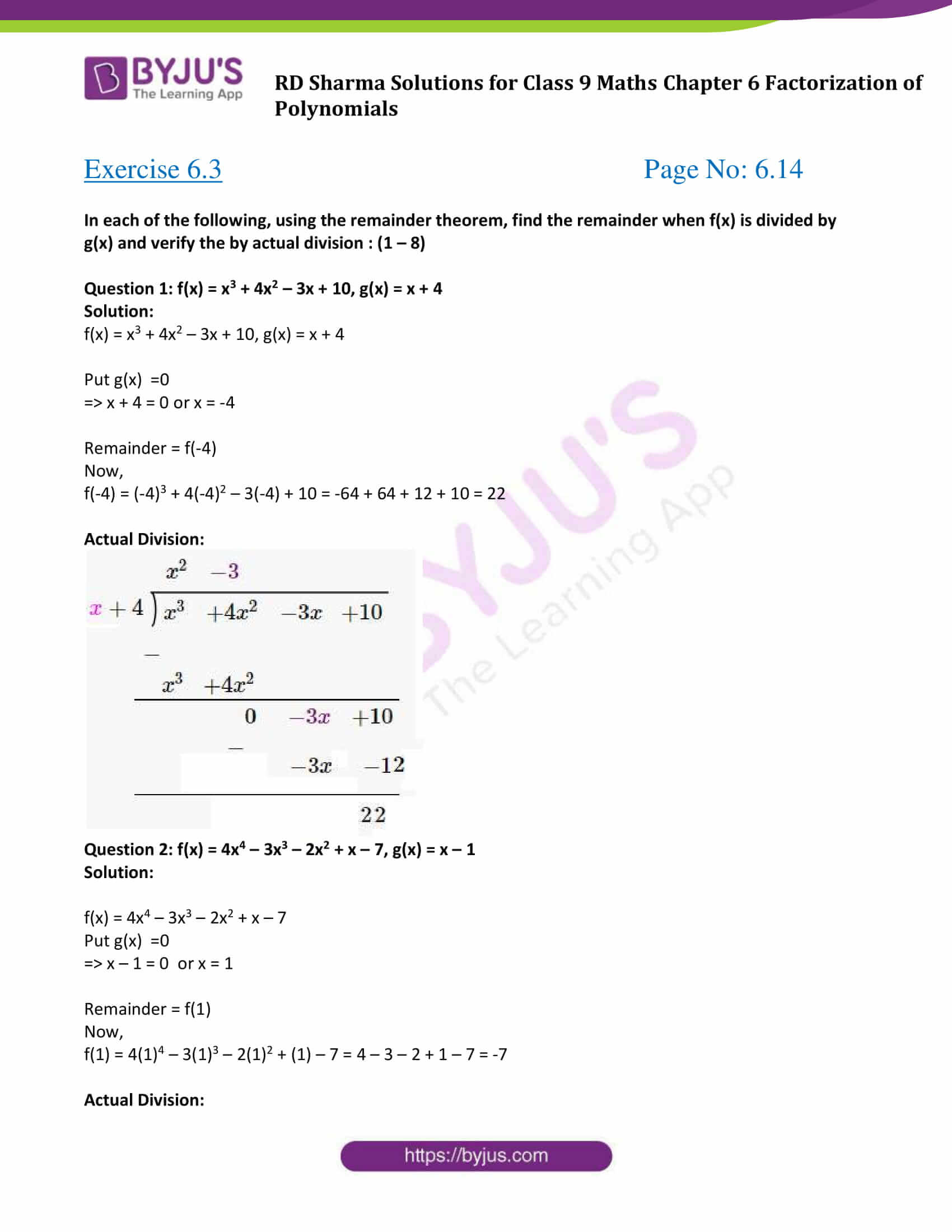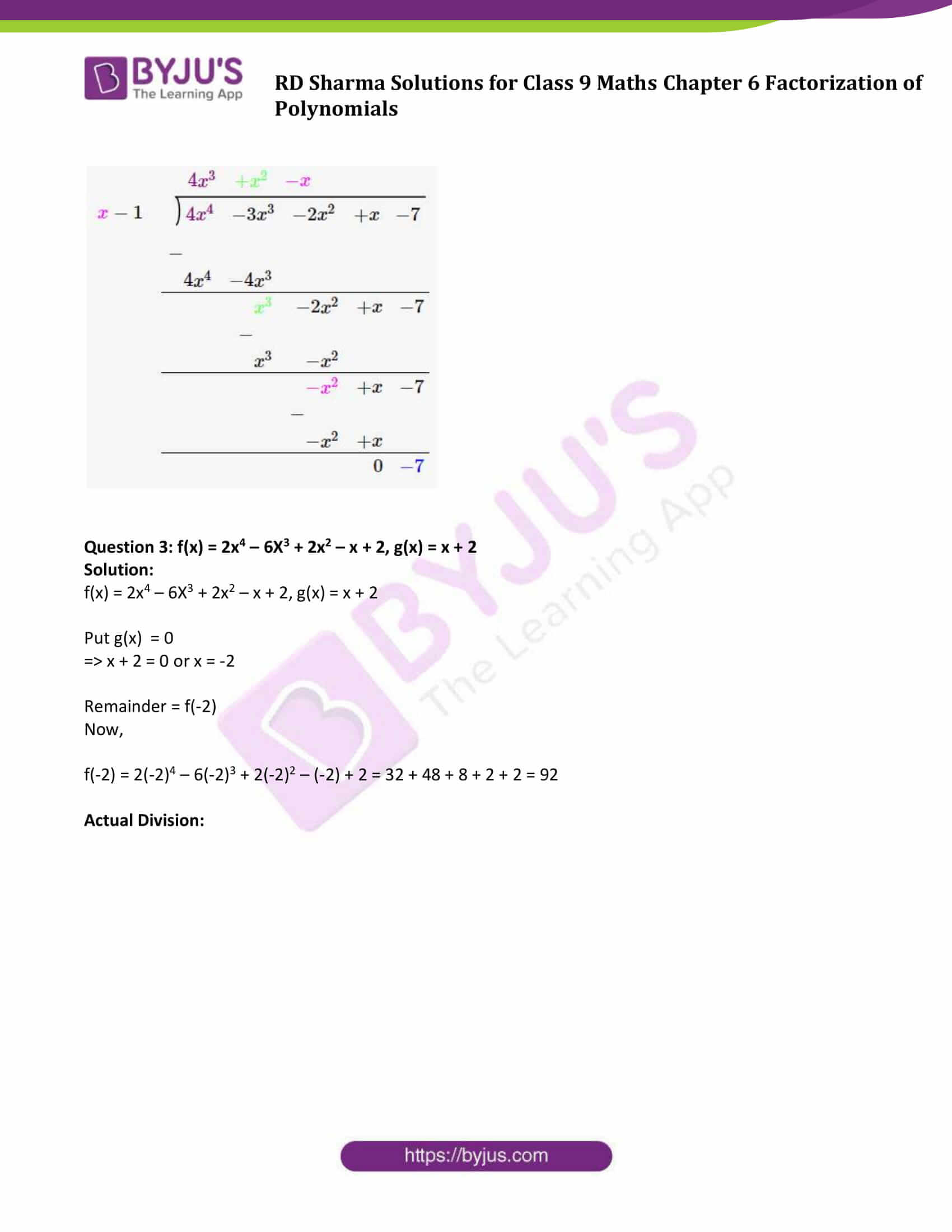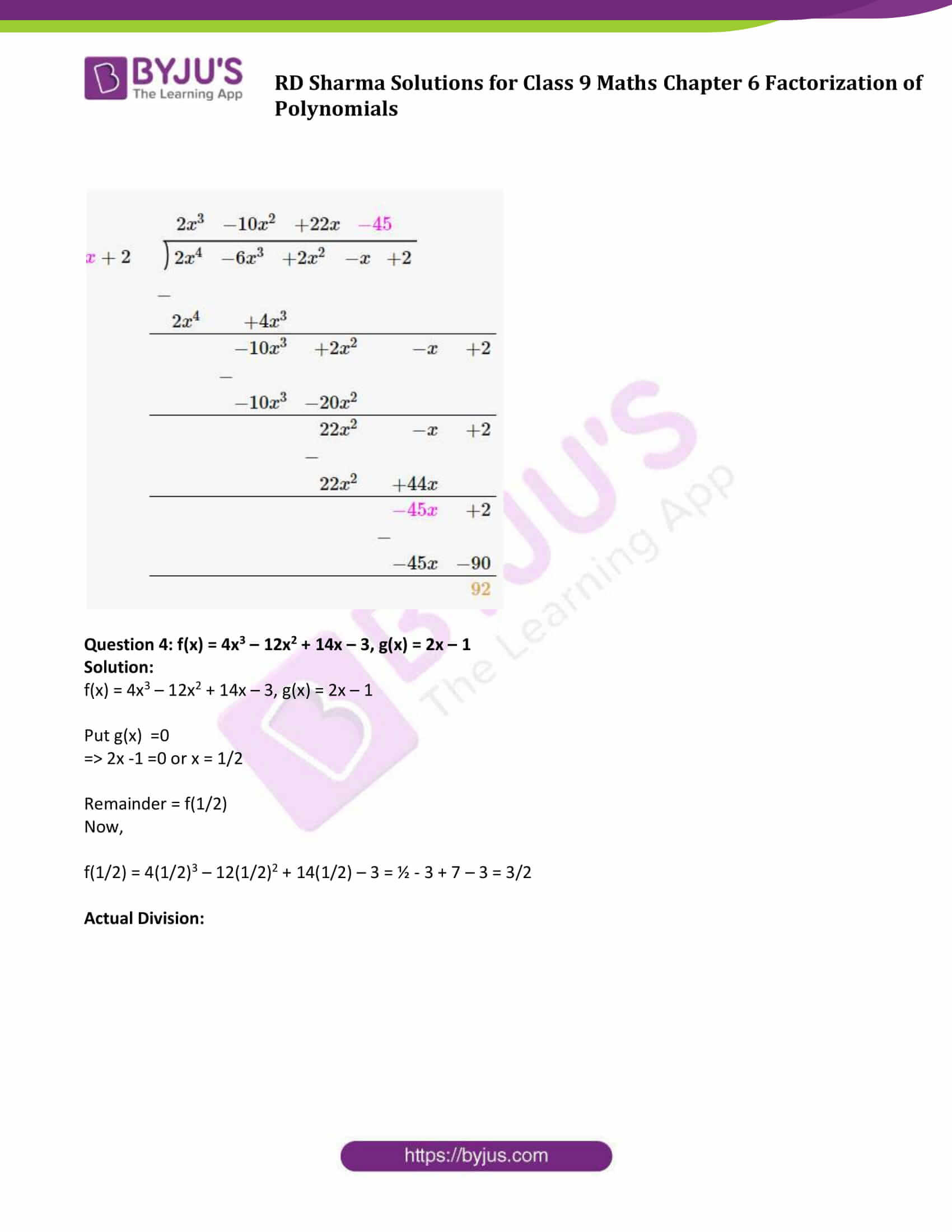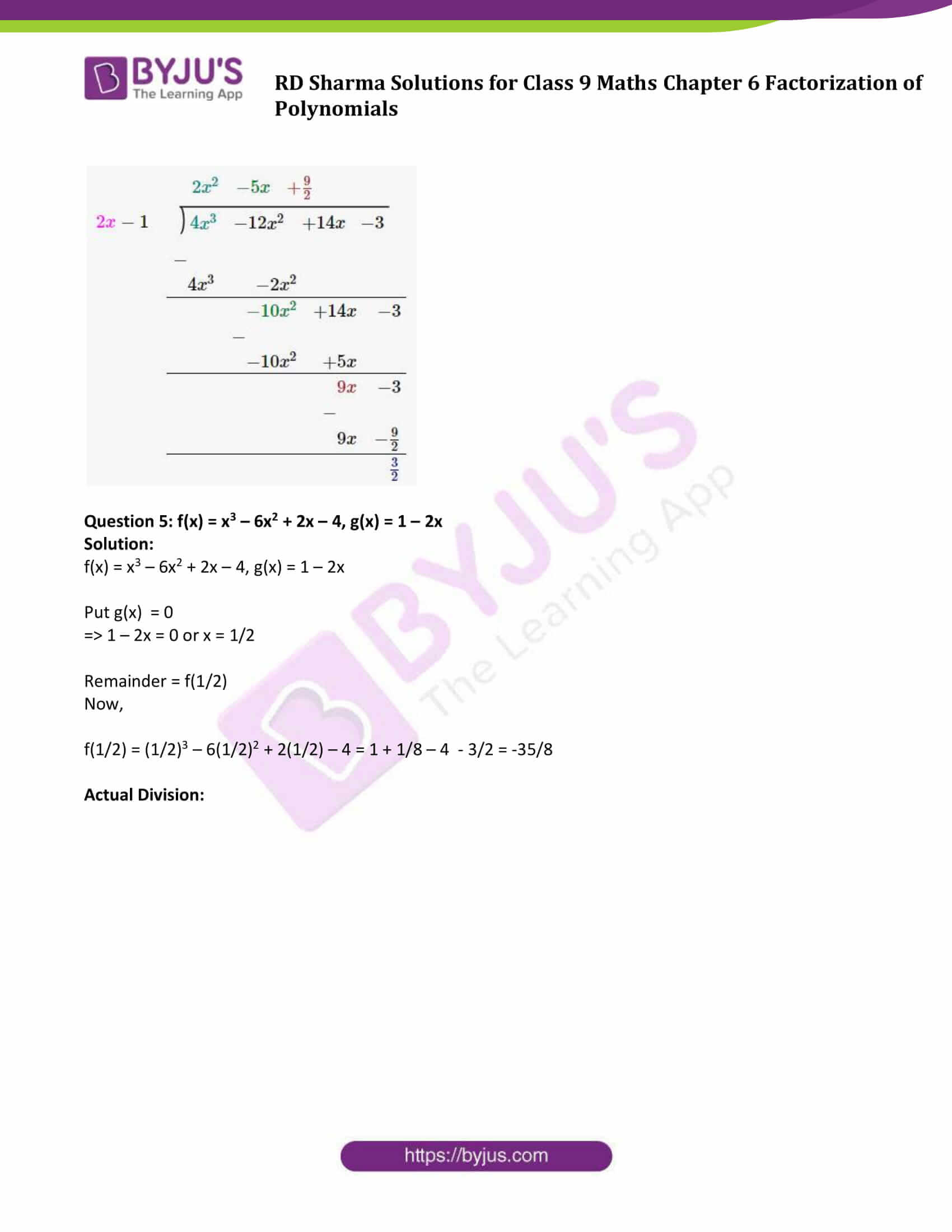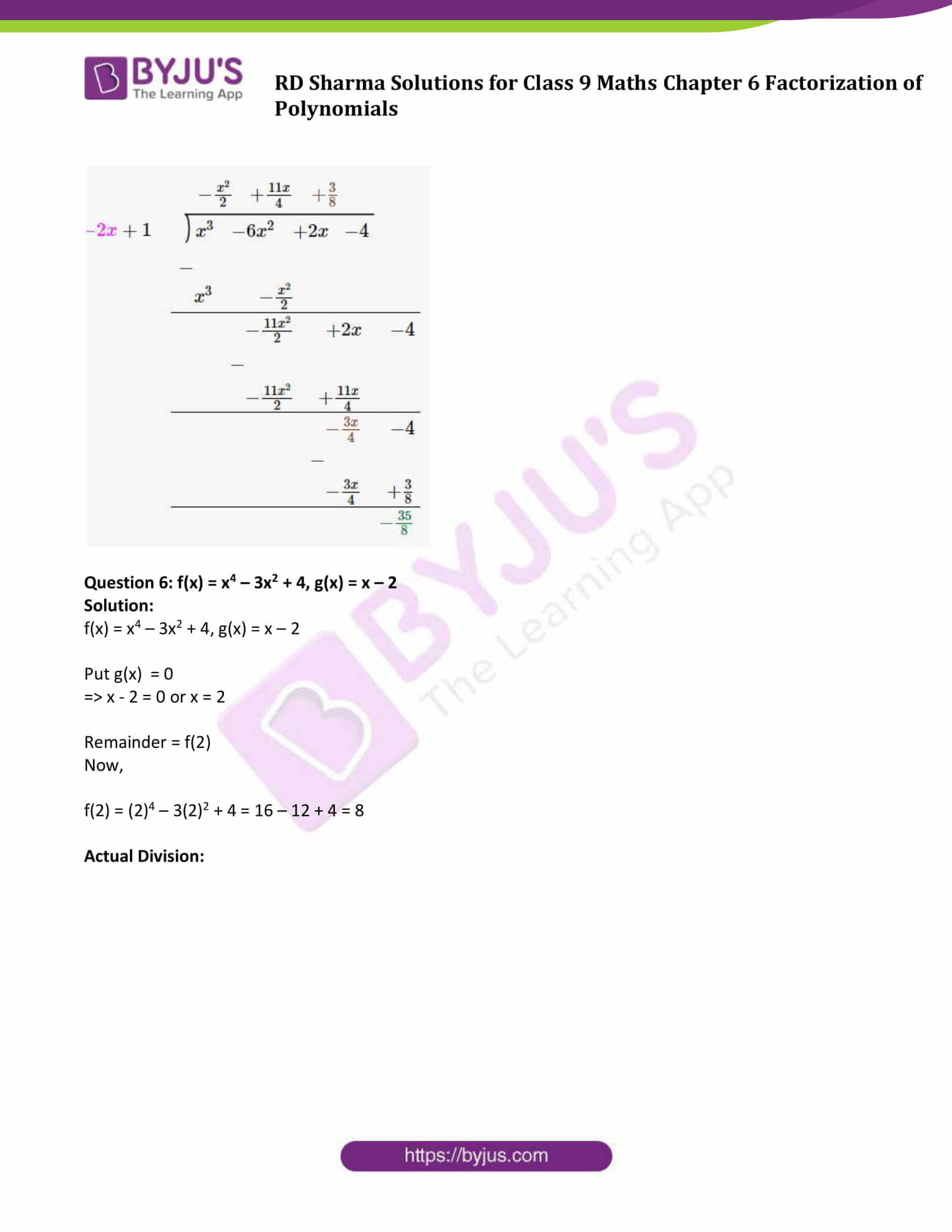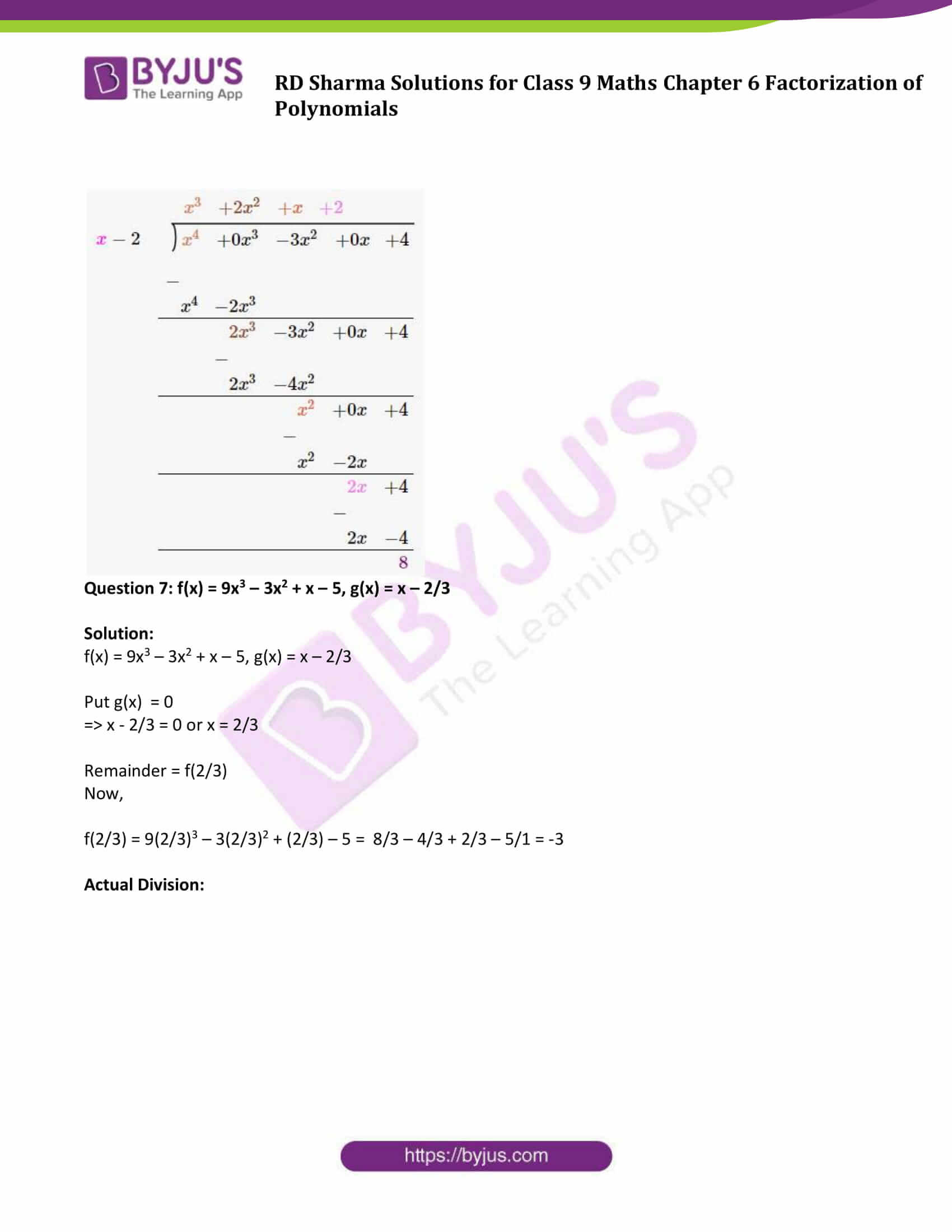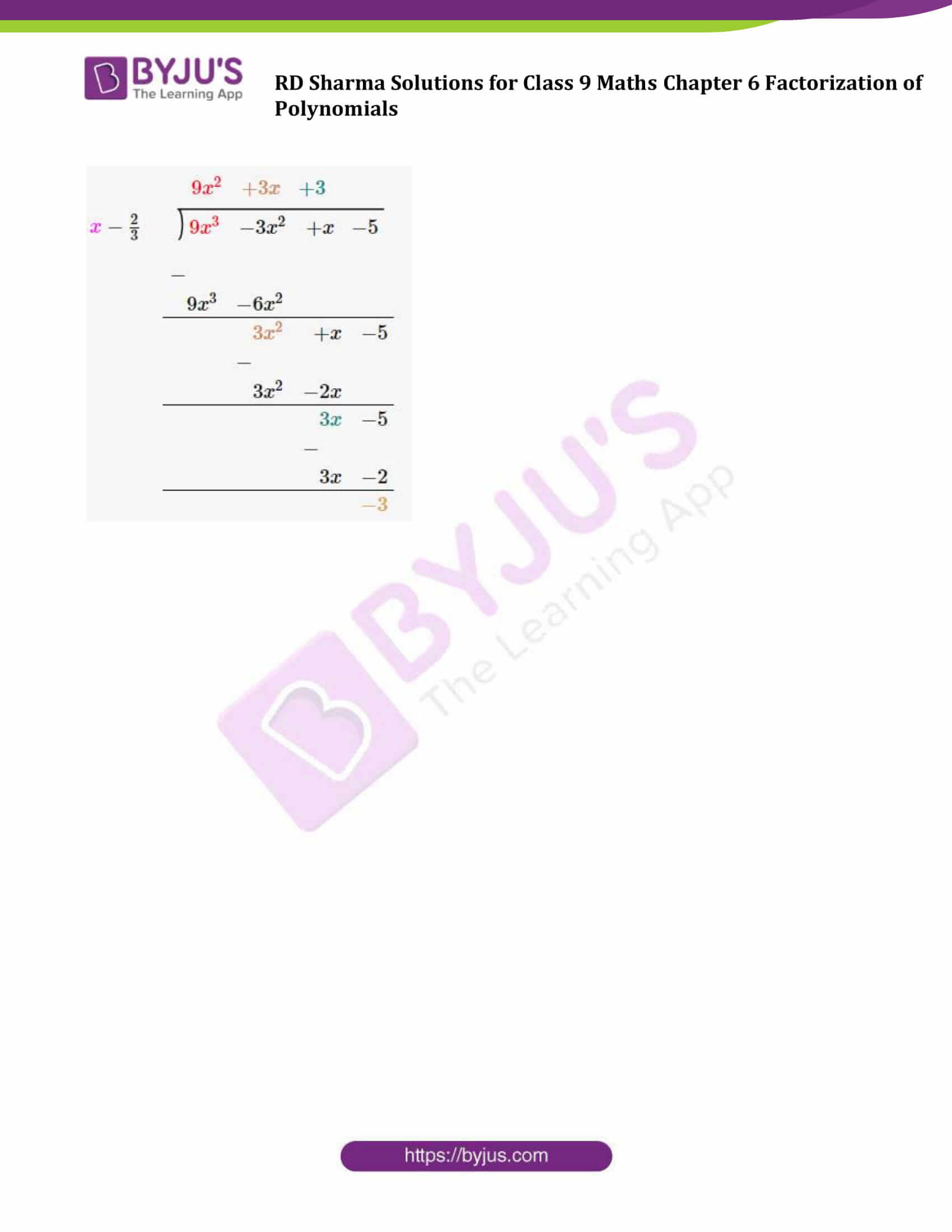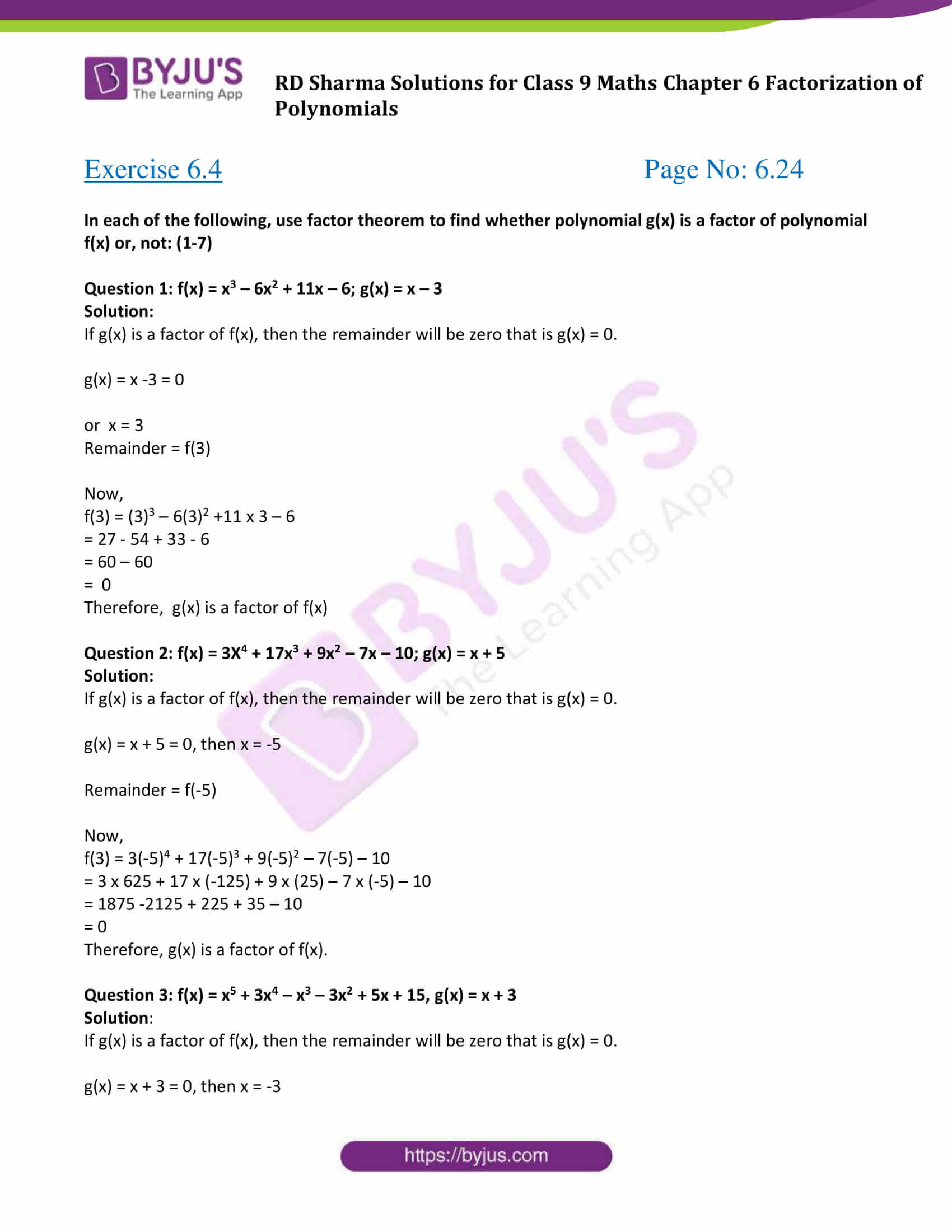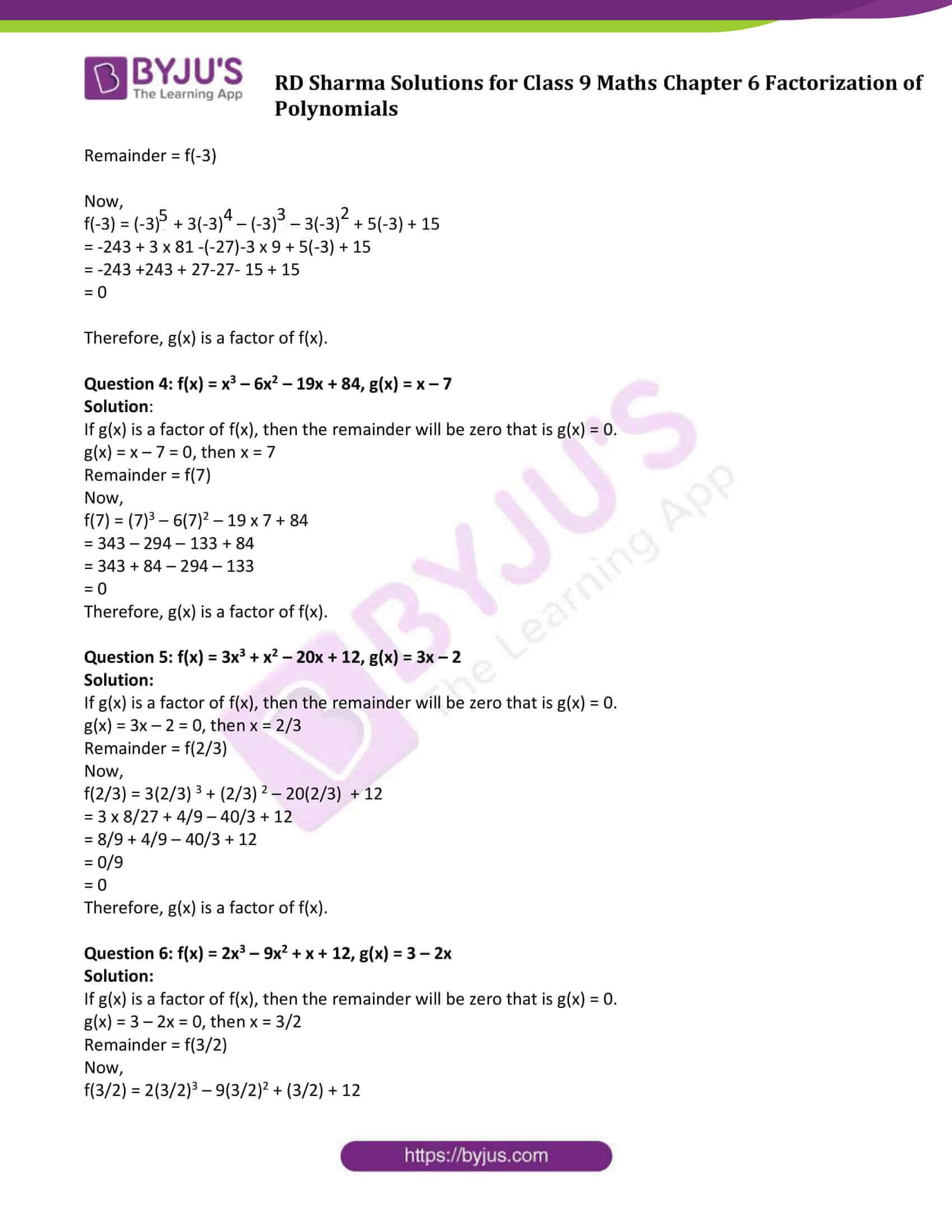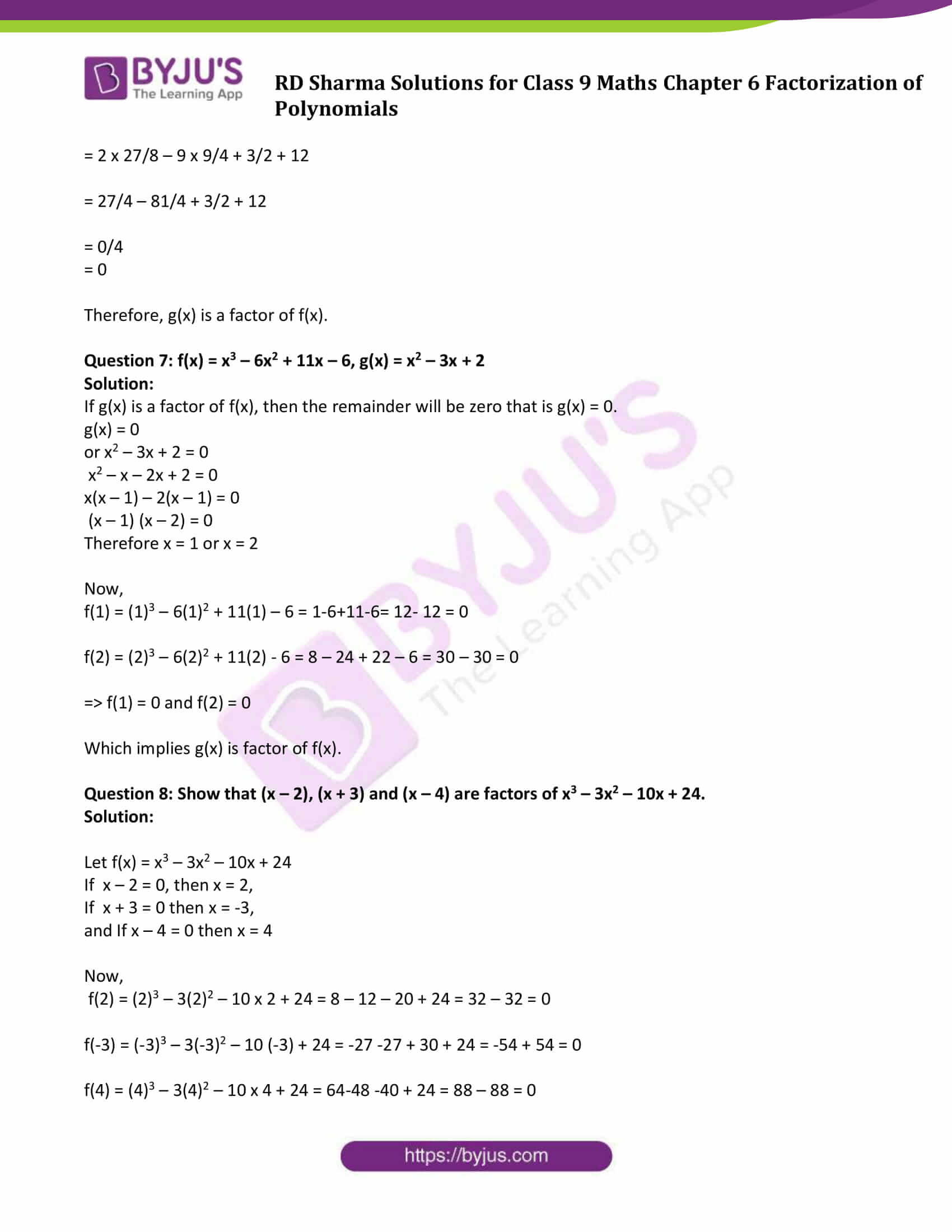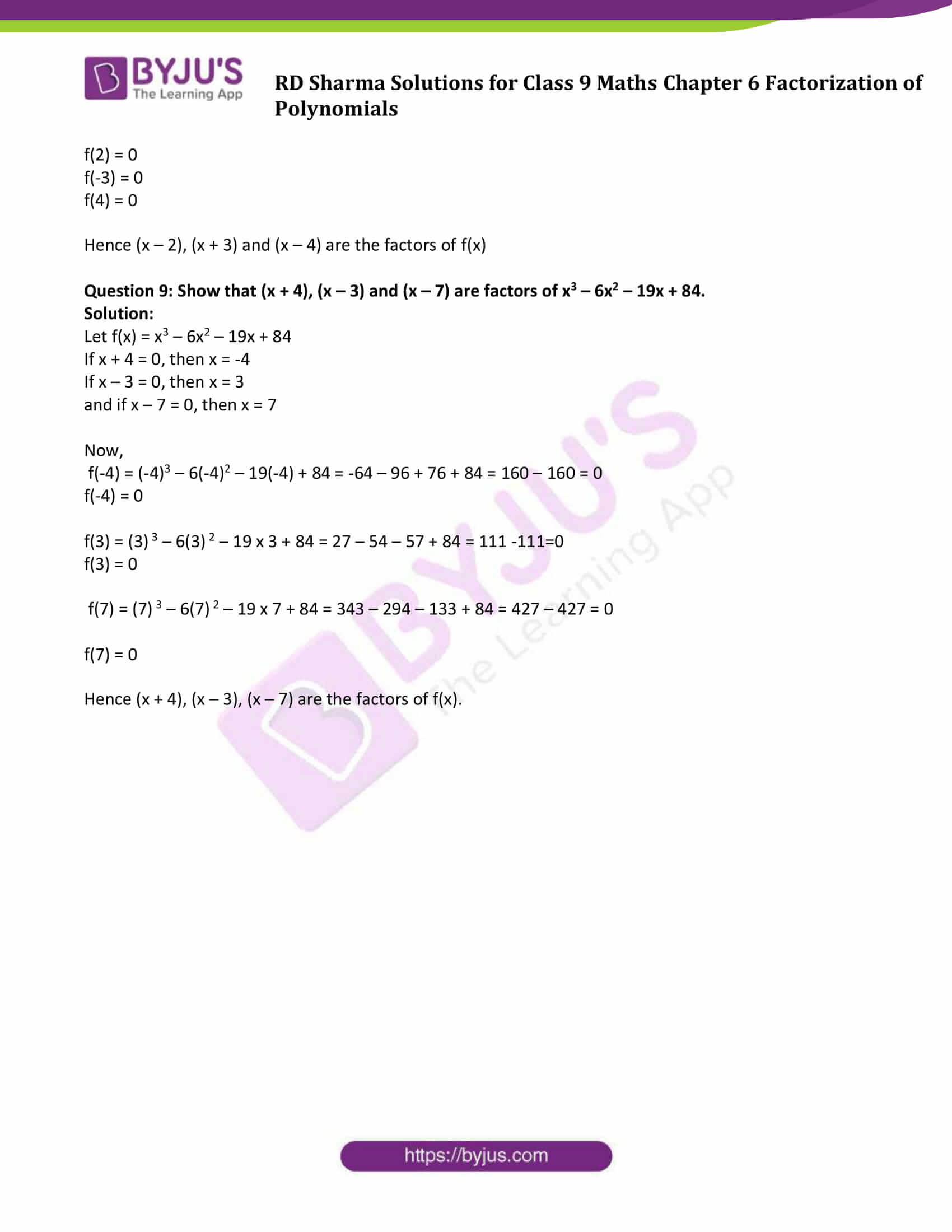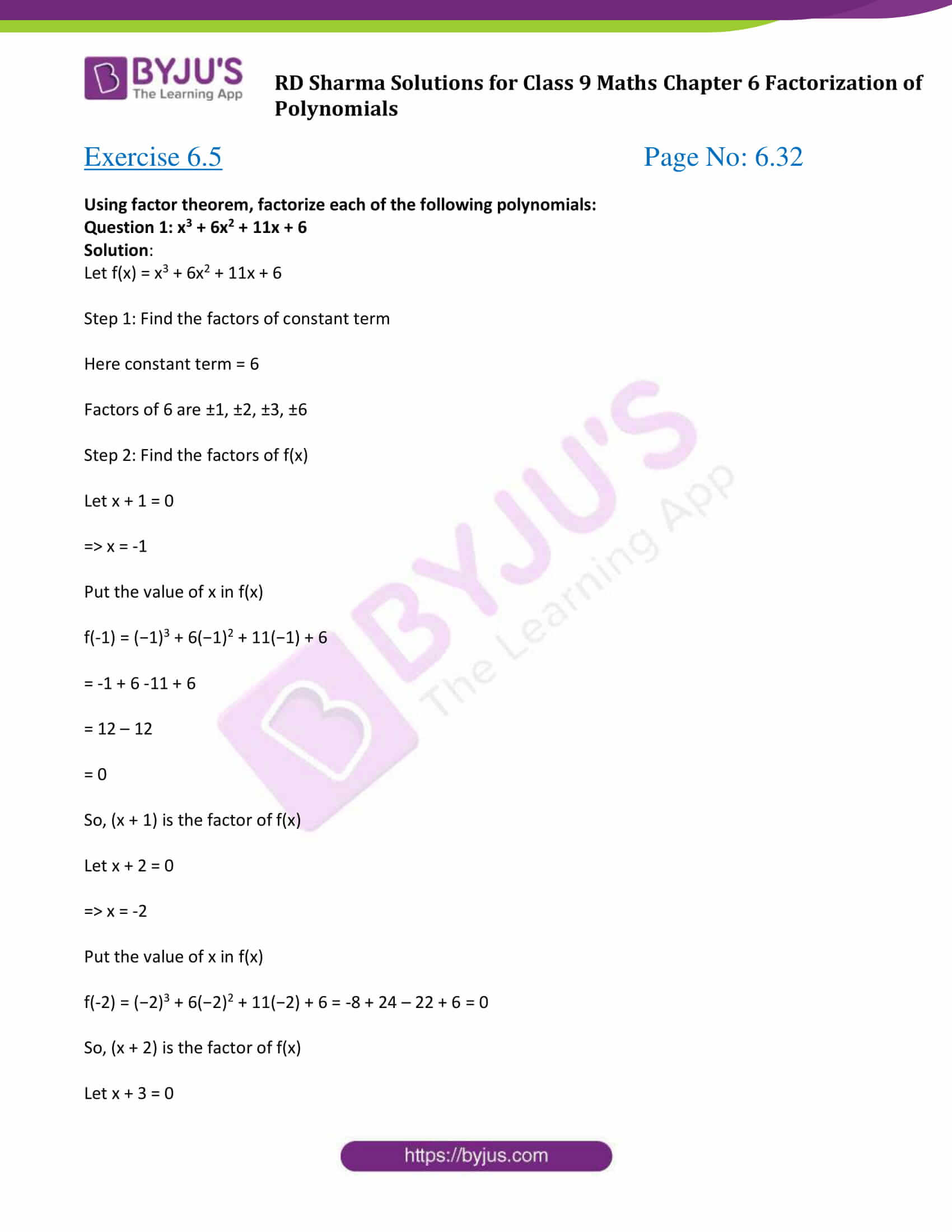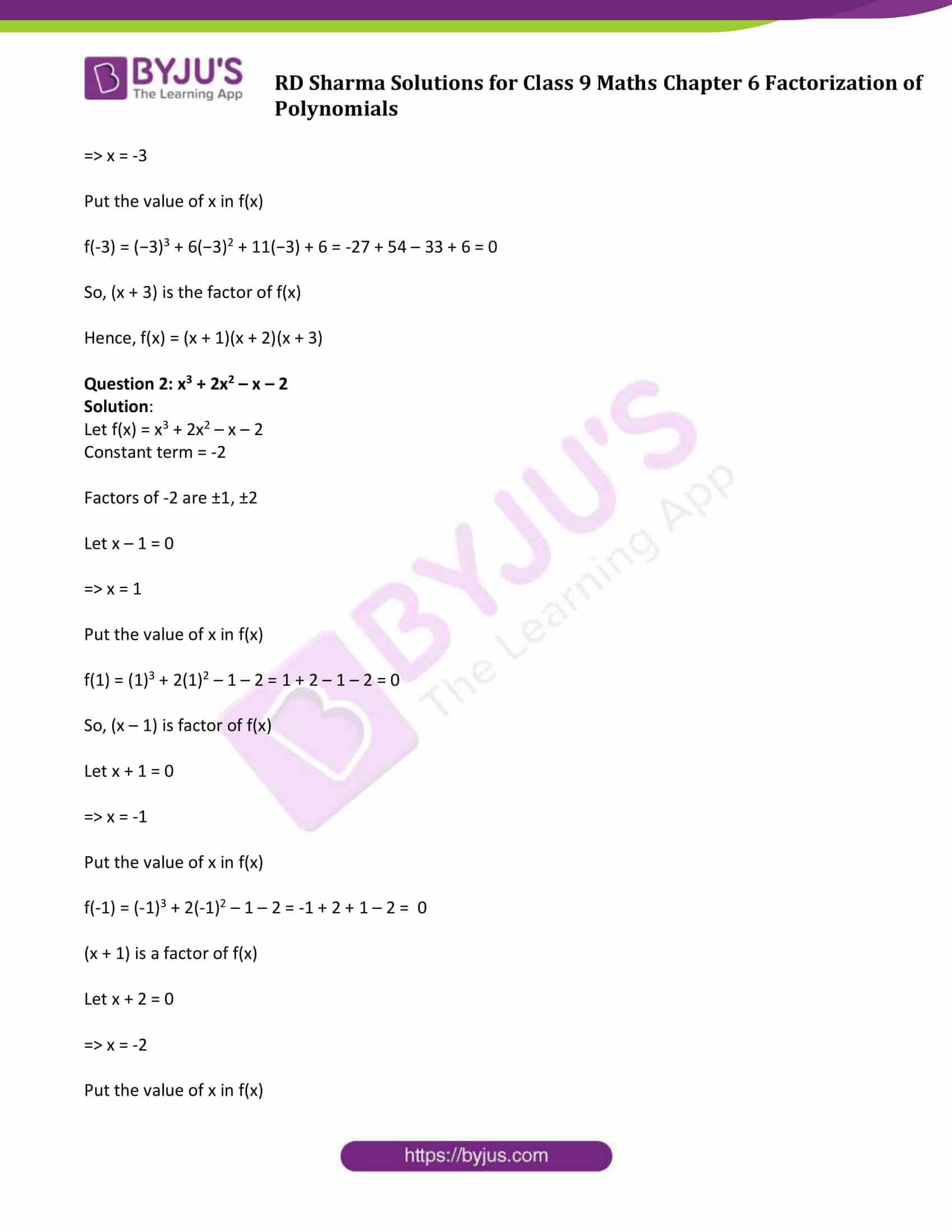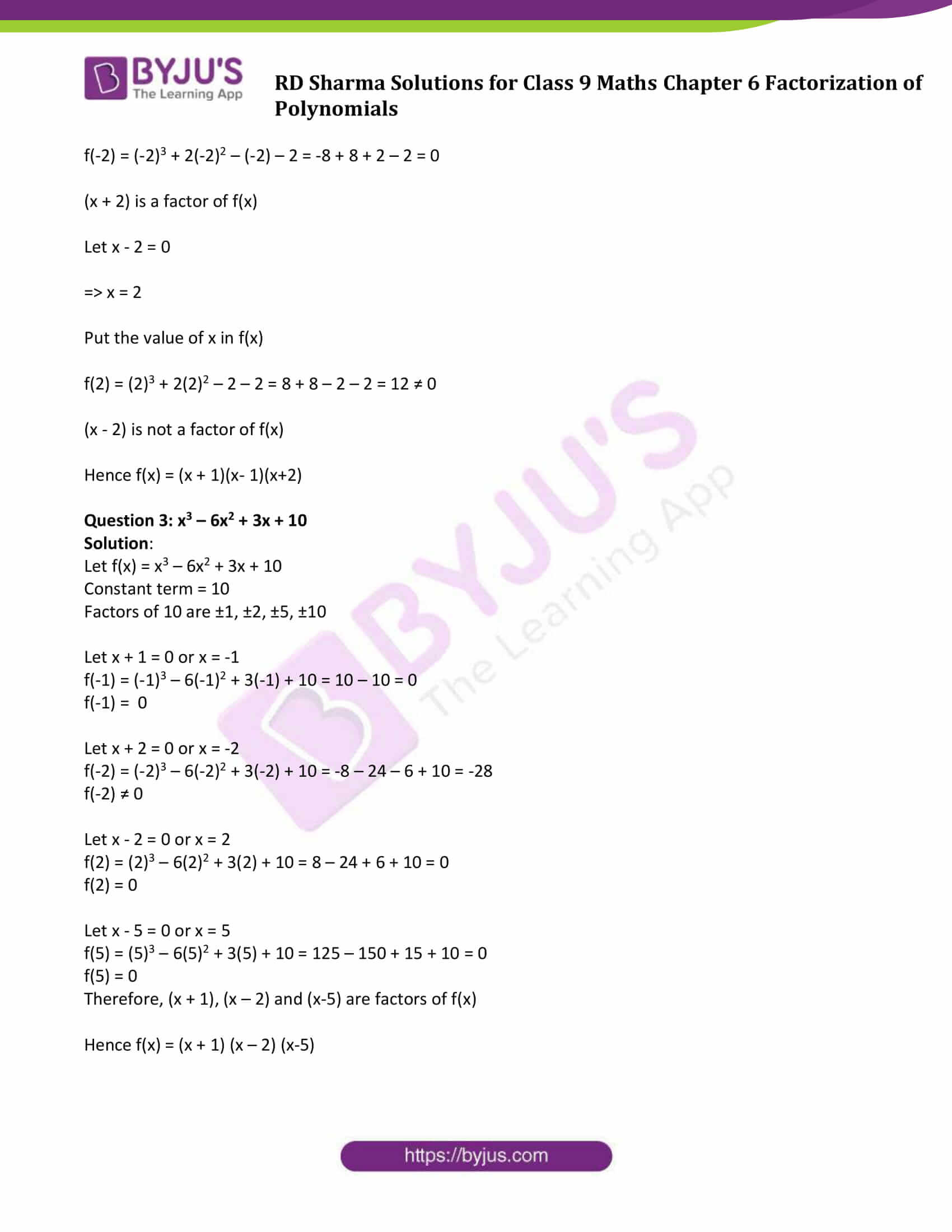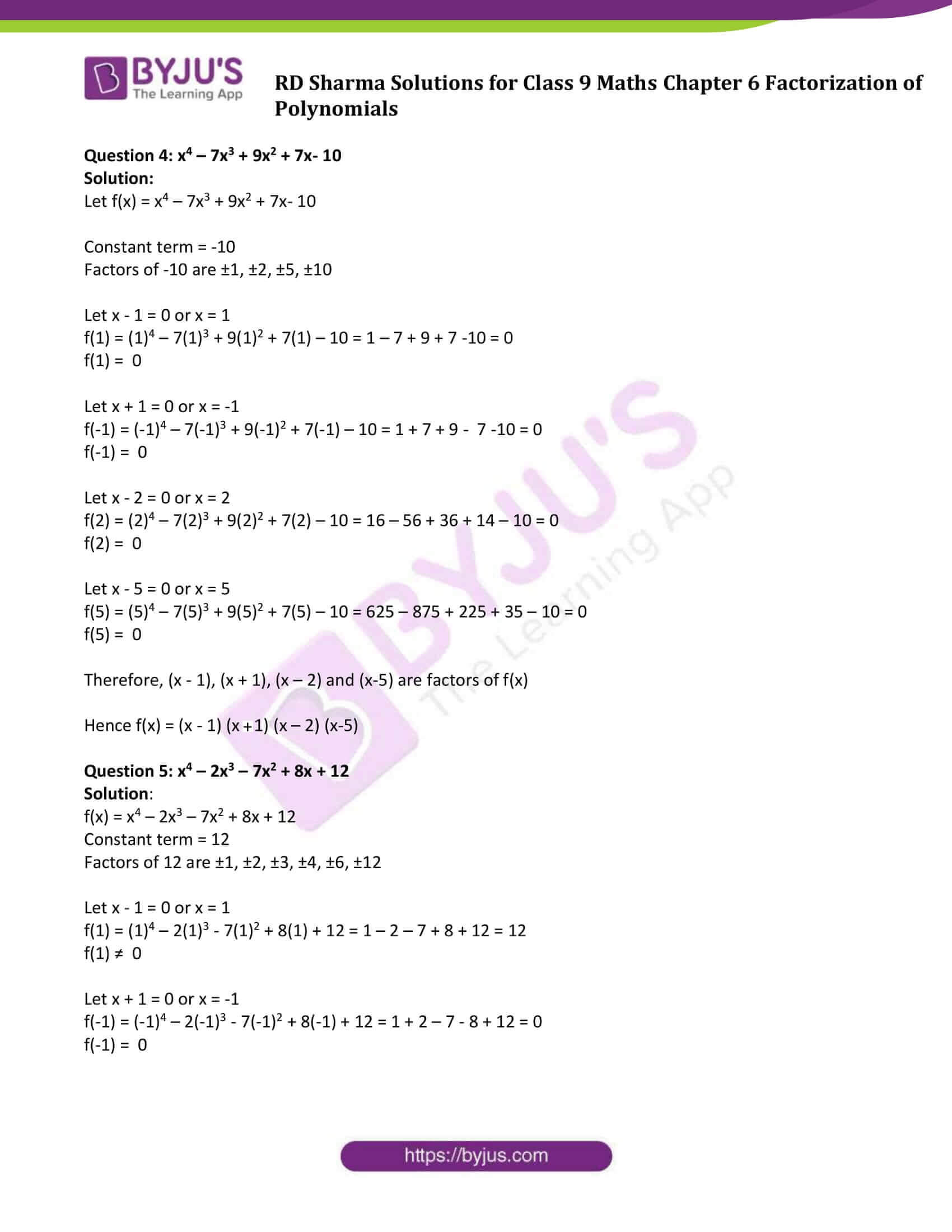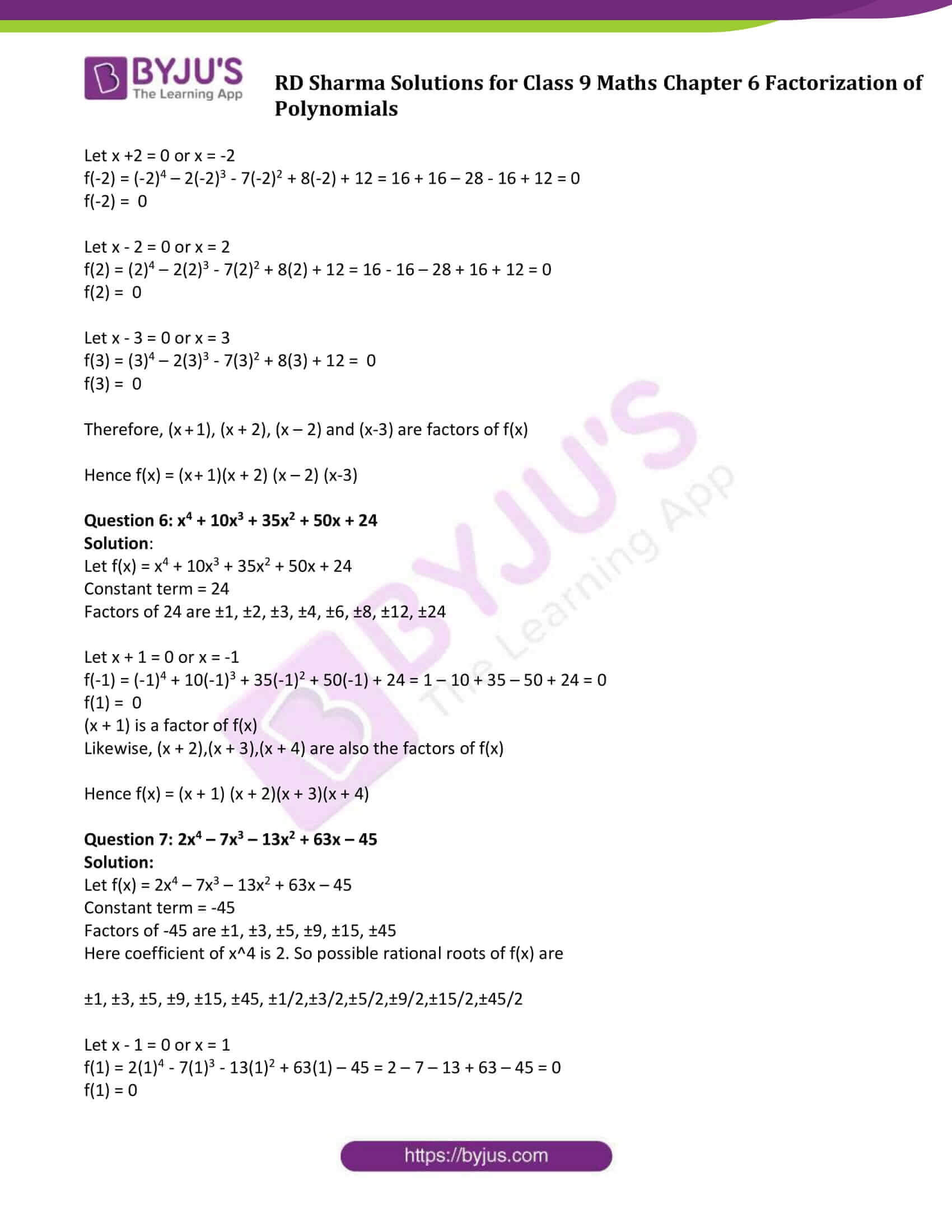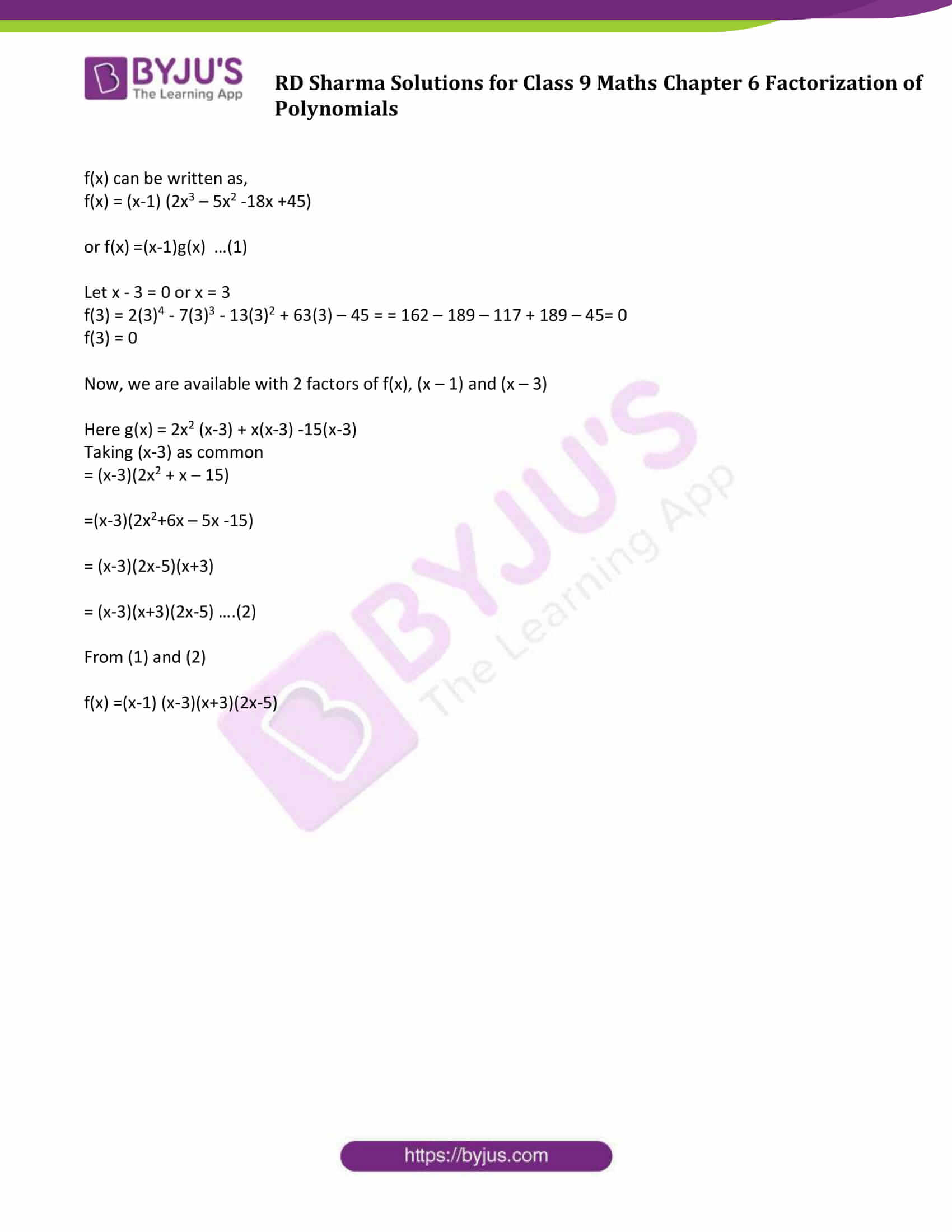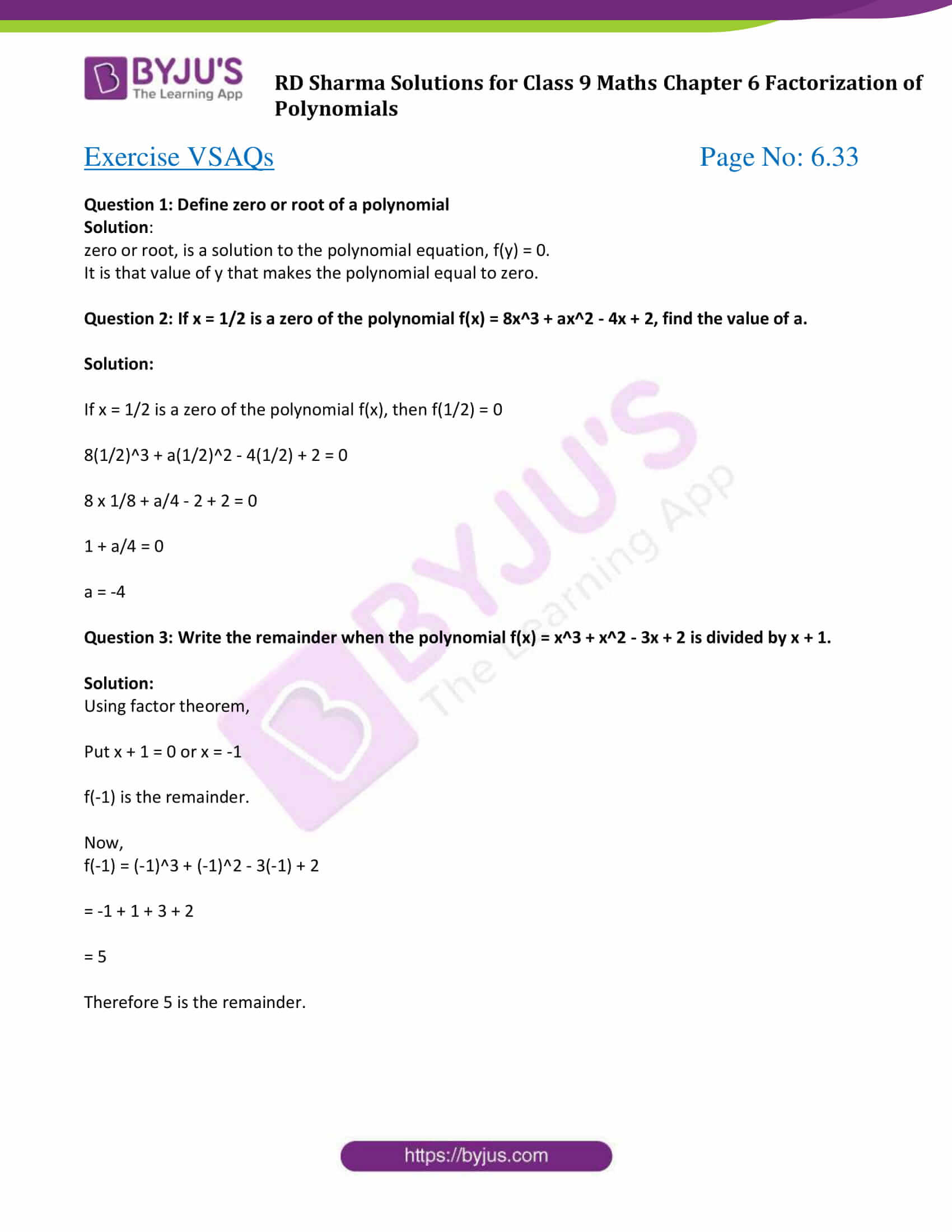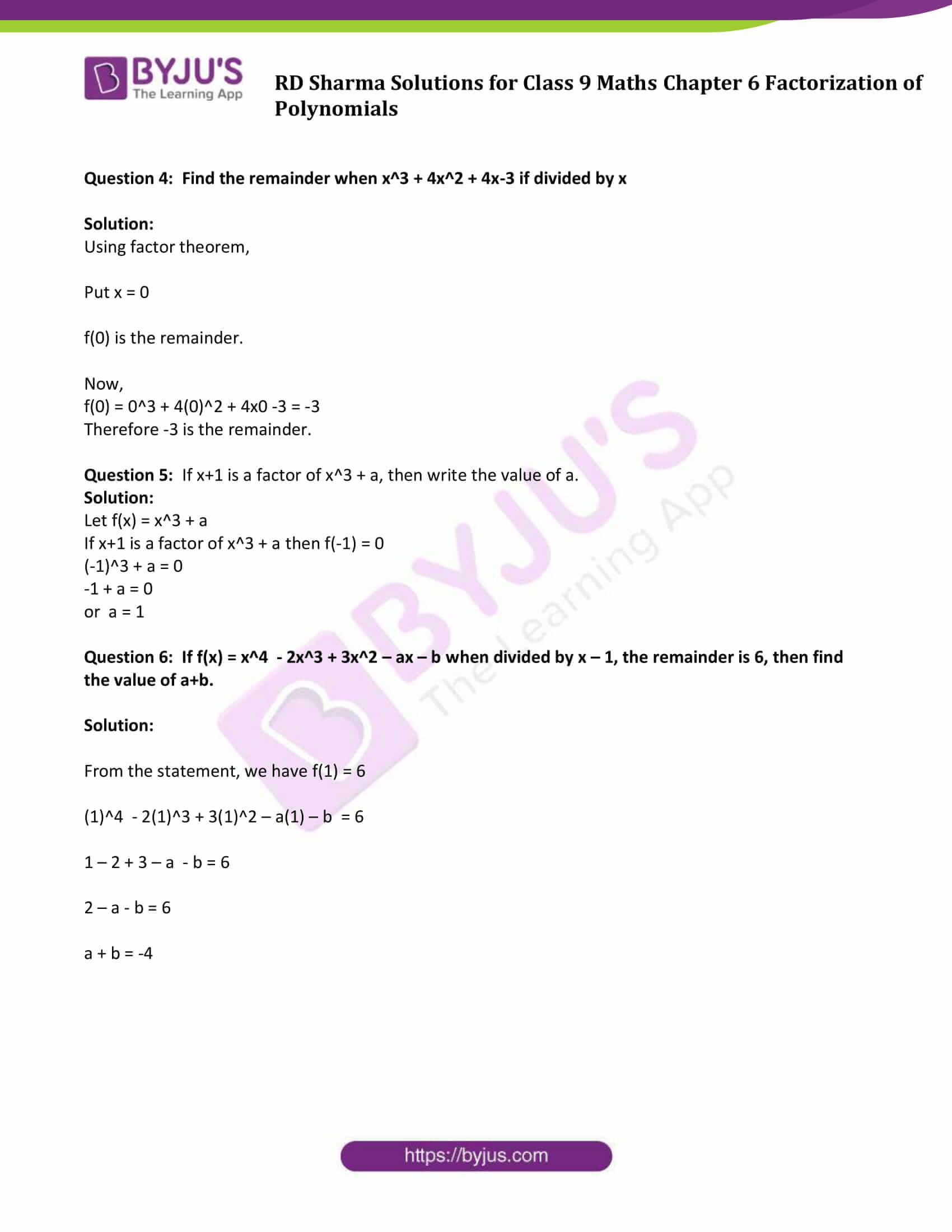### Exercise 6.1 Page No: 6.2

Question 1: Which of the following expressions are polynomials in one variable and which are not? State reasons for your answer:

(i) 3x2 – 4x + 15

(ii) y2 + 2√3

(iii) 3√x + √2x

(iv) x – 4/x

(v) x12 + y3 + t50

Solution:

(i) 3x2 – 4x + 15

It is a polynomial of x.

(ii) y2 + 2√3

It is a polynomial of y.

(iii) 3√x + √2x

It is not a polynomial since the exponent of 3√x is a rational term.

(iv) x – 4/x

It is not a polynomial since the exponent of – 4/x is not a positive term.

(v) x12 + y3 + t50

It is a three variable polynomial, x, y and t.

Question 2: Write the coefficient of x2 in each of the following:

(i) 17 – 2x + 7x2

(ii) 9 – 12x + x3

(iii) ∏/6 x2 – 3x + 4

(iv) √3x – 7

Solution:

(i) 17 – 2x + 7x2

Coefficient of x2 = 7

(ii) 9 – 12x + x3

Coefficient of x2 =0

(iii) ∏/6 x2 – 3x + 4

Coefficient of x2 = ∏/6

(iv) √3x – 7

Coefficient of x2 = 0

Question 3: Write the degrees of each of the following polynomials:

(i) 7x3 + 4x2 – 3x + 12

(ii) 12 – x + 2x3

(iii) 5y – √2

(iv) 7

(v) 0

Solution:

As we know, degree is the highest power in the polynomial

(i) Degree of the polynomial 7x3 + 4x2 – 3x + 12 is 3

(ii) Degree of the polynomial 12 – x + 2x3 is 3

(iii) Degree of the polynomial 5y – √2 is 1

(iv) Degree of the polynomial 7 is 0

(v) Degree of the polynomial 0 is undefined.

Question 4: Classify the following polynomials as linear, quadratic, cubic and biquadratic polynomials:

(i) x + x2 + 4

(ii) 3x – 2

(iii) 2x + x2

(iv) 3y

(v) t2 + 1

(vi) 7t4 + 4t3 + 3t – 2

Solution:

(i) x + x2 + 4: It is a quadratic polynomial as its degree is 2.

(ii) 3x – 2 : It is a linear polynomial as its degree is 1.

(iii) 2x + x2: It is a quadratic polynomial as its degree is 2.

(iv) 3y: It is a linear polynomial as its degree is 1.

(v) t2+ 1: It is a quadratic polynomial as its degree is 2.

(vi) 7t4 + 4t3 + 3t – 2: It is a biquadratic polynomial as its degree is 4.

### Exercise 6.2 Page No: 6.8

Question 1: If f(x) = 2x3 – 13x2 + 17x + 12, find

(i) f (2)

(ii) f (-3)

(iii) f(0)

Solution:

f(x) = 2x3 – 13x2 + 17x + 12

(i) f(2) = 2(2)3 – 13(2) 2 + 17(2) + 12

= 2 x 8 – 13 x 4 + 17 x 2 + 12

= 16 – 52 + 34 + 12

= 62 – 52

= 10

(ii) f(-3) = 2(-3)3 – 13(-3) 2 + 17 x (-3) + 12

= 2 x (-27) – 13 x 9 + 17 x (-3) + 12

= -54 – 117 -51 + 12

= -222 + 12

= -210

(iii) f(0) = 2 x (0)3 – 13(0) 2 + 17 x 0 + 12

= 0-0 + 0+ 12

= 12

Question 2: Verify whether the indicated numbers are zeros of the polynomials corresponding to them in the following cases:

(i) f(x) = 3x + 1, x = −1/3

(ii) f(x) = x2 – 1, x = 1,−1

(iii) g(x) = 3x2 – 2 , x = 2/√3 , −2/√3

(iv) p(x) = x3 – 6x2 + 11x – 6 , x = 1, 2, 3

(v) f(x) = 5x – π, x = 4/5

(vi) f(x) = x2 , x = 0

(vii) f(x) = lx + m, x = −m/l

(viii) f(x) = 2x + 1, x = 1/2

Solution:

(i) f(x) = 3x + 1, x = −1/3

f(x) = 3x + 1

Substitute x = −1/3 in f(x)

f( −1/3) = 3(−1/3) + 1

= -1 + 1

= 0

Since, the result is 0, so x = −1/3 is the root of 3x + 1

(ii) f(x) = x2 – 1, x = 1,−1

f(x) = x2 – 1

Given that x = (1 , -1)

Substitute x = 1 in f(x)

f(1) = 12 – 1

= 1 – 1

= 0

Now, substitute x = (-1) in f(x)

f(-1) = (−1)2 – 1

= 1 – 1

= 0

Since , the results when x = 1 and x = -1 are 0, so (1 , -1) are the roots of the polynomial f(x) = x2 – 1

(iii) g(x) = 3x2 – 2 , x = 2/√3 , −2/√3

g(x) = 3x2 – 2

Substitute x = 2/√3 in g(x)

g(2/√3) = 3(2/√3)2 – 2

= 3(4/3) – 2

= 4 – 2

= 2 ≠ 0

Now, Substitute x = −2/√3 in g(x)

g(2/√3) = 3(-2/√3)2 – 2

= 3(4/3) – 2

= 4 – 2

= 2 ≠ 0

Since, the results when x = 2/√3 and x = −2/√3) are not 0. Therefore (2/√3 , −2/√3 ) are not zeros of 3x2–2.

(iv) p(x) = x3 – 6x2 + 11x – 6 , x = 1, 2, 3

p(1) = 13 – 6(1)2 + 11x 1 – 6 = 1 – 6 + 11 – 6 = 0

p(2) = 23 – 6(2)2 + 11×2 – 6 = 8 – 24 + 22 – 6 = 0

p(3) = 33 – 6(3)2 + 11×3 – 6 = 27 – 54 + 33 – 6 = 0

Therefore, x = 1, 2, 3 are zeros of p(x).

(v) f(x) = 5x – π, x = 4/5

f(4/5) = 5 x 4/5 – π = 4 – π ≠ 0

Therefore, x = 4/5 is not a zeros of f(x).

(vi) f(x) = x2 , x = 0

f(0) = 02 = 0

Therefore, x = 0 is a zero of f(x).

(vii) f(x) = lx + m, x = −m/l

f(−m/l) = l x −m/l + m = -m + m = 0

Therefore, x = −m/l is a zero of f(x).

(viii) f(x) = 2x + 1, x = ½

f(1/2) = 2x 1/2 + 1 = 1 + 1 = 2 ≠ 0

Therefore, x = ½ is not a zero of f(x).

### Exercise 6.3 Page No: 6.14

In each of the following, using the remainder theorem, find the remainder when f(x) is divided by g(x) and verify the by actual division : (1 – 8)

Question 1: f(x) = x3 + 4x2 – 3x + 10, g(x) = x + 4

Solution:

f(x) = x3 + 4x2 – 3x + 10, g(x) = x + 4

Put g(x) =0

⇒ x + 4 = 0 or x = -4

Remainder = f(-4)

Now,

f(-4) = (-4)3 + 4(-4)2 – 3(-4) + 10 = -64 + 64 + 12 + 10 = 22

Actual Division: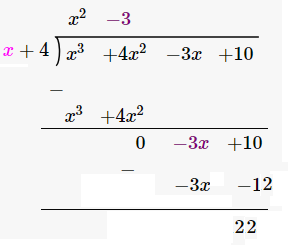Question 2: f(x) = 4x4 – 3x3 – 2x2 + x – 7, g(x) = x – 1

Solution:

f(x) = 4x4 – 3x3 – 2x2 + x – 7

Put g(x) =0

⇒ x – 1 = 0 or x = 1

Remainder = f(1)

Now,

f(1) = 4(1)4 – 3(1)3 – 2(1)2 + (1) – 7 = 4 – 3 – 2 + 1 – 7 = -7

Actual Division: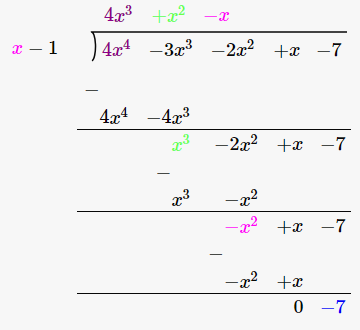Question 3: f(x) = 2x4 – 6X3 + 2x2 – x + 2, g(x) = x + 2

Solution:

f(x) = 2x4 – 6X3 + 2x2 – x + 2, g(x) = x + 2

Put g(x) = 0

⇒ x + 2 = 0 or x = -2

Remainder = f(-2)

Now,

f(-2) = 2(-2)4 – 6(-2)3 + 2(-2)2 – (-2) + 2 = 32 + 48 + 8 + 2 + 2 = 92

Actual Division: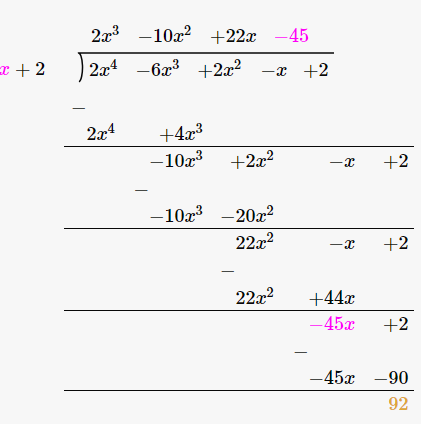Question 4: f(x) = 4x3 – 12x2 + 14x – 3, g(x) = 2x – 1

Solution:

f(x) = 4x3 – 12x2 + 14x – 3, g(x) = 2x – 1

Put g(x) =0

⇒ 2x -1 =0 or x = 1/2

Remainder = f(1/2)

Now,

f(1/2) = 4(1/2)3 – 12(1/2)2 + 14(1/2) – 3 = ½ – 3 + 7 – 3 = 3/2

Actual Division: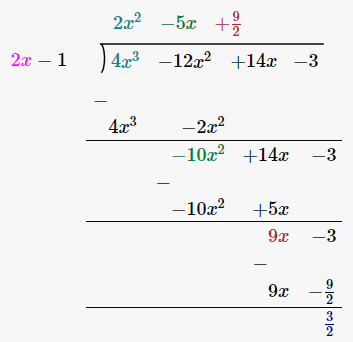Question 5: f(x) = x3 – 6x2 + 2x – 4, g(x) = 1 – 2x

Solution:

f(x) = x3 – 6x2 + 2x – 4, g(x) = 1 – 2x

Put g(x) = 0

⇒ 1 – 2x = 0 or x = 1/2

Remainder = f(1/2)

Now,

f(1/2) = (1/2)3 – 6(1/2)2 + 2(1/2) – 4 = 1 + 1/8 – 4 – 3/2 = -35/8

Actual Division:Question 6: f(x) = x4 – 3x2 + 4, g(x) = x – 2

Solution:

f(x) = x4 – 3x2 + 4, g(x) = x – 2

Put g(x) = 0

⇒ x – 2 = 0 or x = 2

Remainder = f(2)

Now,

f(2) = (2)4 – 3(2)2 + 4 = 16 – 12 + 4 = 8

Actual Division: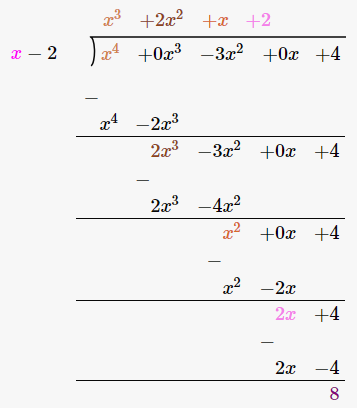Question 7: f(x) = 9x3 – 3x2 + x – 5, g(x) = x – 2/3

Solution:

f(x) = 9x3 – 3x2 + x – 5, g(x) = x – 2/3

Put g(x) = 0

⇒ x – 2/3 = 0 or x = 2/3

Remainder = f(2/3)

Now,

f(2/3) = 9(2/3)3 – 3(2/3)2 + (2/3) – 5 = 8/3 – 4/3 + 2/3 – 5/1 = -3

Actual Division: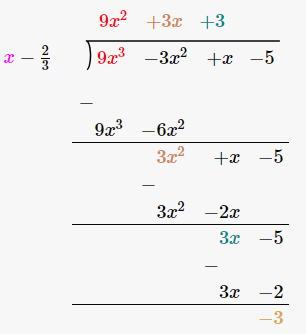### Exercise 6.4 Page No: 6.24

In each of the following, use factor theorem to find whether polynomial g(x) is a factor of polynomial f(x) or, not: (1-7)

Question 1: f(x) = x3 – 6x2 + 11x – 6; g(x) = x – 3

Solution:

If g(x) is a factor of f(x), then the remainder will be zero that is g(x) = 0.

g(x) = x -3 = 0

or x = 3

Remainder = f(3)

Now,

f(3) = (3)3 – 6(3)2 +11 x 3 – 6

= 27 – 54 + 33 – 6

= 60 – 60

= 0

Therefore, g(x) is a factor of f(x)

Question 2: f(x) = 3X4 + 17x3 + 9x2 – 7x – 10; g(x) = x + 5

Solution:

If g(x) is a factor of f(x), then the remainder will be zero that is g(x) = 0.

g(x) = x + 5 = 0, then x = -5

Remainder = f(-5)

Now,

f(3) = 3(-5)4 + 17(-5)3 + 9(-5)2 – 7(-5) – 10

= 3 x 625 + 17 x (-125) + 9 x (25) – 7 x (-5) – 10

= 1875 -2125 + 225 + 35 – 10

= 0

Therefore, g(x) is a factor of f(x).

Question 3: f(x) = x5 + 3x4 – x3 – 3x2 + 5x + 15, g(x) = x + 3

Solution:

If g(x) is a factor of f(x), then the remainder will be zero that is g(x) = 0.

g(x) = x + 3 = 0, then x = -3

Remainder = f(-3)

Now,

f(-3) = (-3)5 + 3(-3)4 – (-3)3 – 3(-3)2 + 5(-3) + 15

= -243 + 3 x 81 -(-27)-3 x 9 + 5(-3) + 15

= -243 +243 + 27-27- 15 + 15

= 0

Therefore, g(x) is a factor of f(x).

Question 4: f(x) = x3 – 6x2 – 19x + 84, g(x) = x – 7

Solution:

If g(x) is a factor of f(x), then the remainder will be zero that is g(x) = 0.

g(x) = x – 7 = 0, then x = 7

Remainder = f(7)

Now,

f(7) = (7)3 – 6(7)2 – 19 x 7 + 84

= 343 – 294 – 133 + 84

= 343 + 84 – 294 – 133

= 0

Therefore, g(x) is a factor of f(x).

Question 5: f(x) = 3x3 + x2 – 20x + 12, g(x) = 3x – 2

Solution:

If g(x) is a factor of f(x), then the remainder will be zero that is g(x) = 0.

g(x) = 3x – 2 = 0, then x = 2/3

Remainder = f(2/3)

Now,

f(2/3) = 3(2/3) 3 + (2/3) 2 – 20(2/3) + 12

= 3 x 8/27 + 4/9 – 40/3 + 12

= 8/9 + 4/9 – 40/3 + 12

= 0/9

= 0

Therefore, g(x) is a factor of f(x).

Question 6: f(x) = 2x3 – 9x2 + x + 12, g(x) = 3 – 2x

Solution:

If g(x) is a factor of f(x), then the remainder will be zero that is g(x) = 0.

g(x) = 3 – 2x = 0, then x = 3/2

Remainder = f(3/2)

Now,

f(3/2) = 2(3/2)3 – 9(3/2)2 + (3/2) + 12

= 2 x 27/8 – 9 x 9/4 + 3/2 + 12

= 27/4 – 81/4 + 3/2 + 12

= 0/4

= 0

Therefore, g(x) is a factor of f(x).

Question 7: f(x) = x3 – 6x2 + 11x – 6, g(x) = x2 – 3x + 2

Solution:

If g(x) is a factor of f(x), then the remainder will be zero that is g(x) = 0.

g(x) = 0

or x2 – 3x + 2 = 0

x2 – x – 2x + 2 = 0

x(x – 1) – 2(x – 1) = 0

(x – 1) (x – 2) = 0

Therefore x = 1 or x = 2

Now,

‍f(1) = (1)3 – 6(1)2 + 11(1) – 6 = 1-6+11-6= 12- 12 = 0

f(2) = (2)3 – 6(2)2 + 11(2) – 6 = 8 – 24 + 22 – 6 = 30 – 30 = 0

⇒ f(1) = 0 and f(2) = 0

Which implies g(x) is factor of f(x).

Question 8: Show that (x – 2), (x + 3) and (x – 4) are factors of x3 – 3x2 – 10x + 24.

Solution:

Let f(x) = x3 – 3x2 – 10x + 24

If x – 2 = 0, then x = 2,

If x + 3 = 0 then x = -3,

and If x – 4 = 0 then x = 4

Now,

f(2) = (2)3 – 3(2)2 – 10 x 2 + 24 = 8 – 12 – 20 + 24 = 32 – 32 = 0

f(-3) = (-3)3 – 3(-3)2 – 10 (-3) + 24 = -27 -27 + 30 + 24 = -54 + 54 = 0

f(4) = (4)3 – 3(4)2 – 10 x 4 + 24 = 64-48 -40 + 24 = 88 – 88 = 0

f(2) = 0

f(-3) = 0

f(4) = 0

Hence (x – 2), (x + 3) and (x – 4) are the factors of f(x)

Question 9: Show that (x + 4), (x – 3) and (x – 7) are factors of x3 – 6x2 – 19x + 84.

Solution:

Let f(x) = x3 – 6x2 – 19x + 84

If x + 4 = 0, then x = -4

If x – 3 = 0, then x = 3

and if x – 7 = 0, then x = 7

Now,

f(-4) = (-4)3 – 6(-4)2 – 19(-4) + 84 = -64 – 96 + 76 + 84 = 160 – 160 = 0

f(-4) = 0

f(3) = (3) 3 – 6(3) 2 – 19 x 3 + 84 = 27 – 54 – 57 + 84 = 111 -111=0

f(3) = 0

f(7) = (7) 3 – 6(7) 2 – 19 x 7 + 84 = 343 – 294 – 133 + 84 = 427 – 427 = 0

f(7) = 0

Hence (x + 4), (x – 3), (x – 7) are the factors of f(x).

### Exercise 6.5 Page No: 6.32

Using factor theorem, factorize each of the following polynomials:

Question 1: x3 + 6x2 + 11x + 6

Solution:

Let f(x) = x3 + 6x2 + 11x + 6

Step 1: Find the factors of constant term

Here constant term = 6

Factors of 6 are ±1, ±2, ±3, ±6

Step 2: Find the factors of f(x)

Let x + 1 = 0

⇒ x = -1

Put the value of x in f(x)

f(-1) = (−1)3 + 6(−1)2 + 11(−1) + 6

= -1 + 6 -11 + 6

= 12 – 12

= 0

So, (x + 1) is the factor of f(x)

Let x + 2 = 0

⇒ x = -2

Put the value of x in f(x)

f(-2) = (−2)3 + 6(−2)2 + 11(−2) + 6 = -8 + 24 – 22 + 6 = 0

So, (x + 2) is the factor of f(x)

Let x + 3 = 0

⇒ x = -3

Put the value of x in f(x)

f(-3) = (−3)3 + 6(−3)2 + 11(−3) + 6 = -27 + 54 – 33 + 6 = 0

So, (x + 3) is the factor of f(x)

Hence, f(x) = (x + 1)(x + 2)(x + 3)

Question 2: x3 + 2x2 – x – 2

Solution:

Let f(x) = x3 + 2x2 – x – 2

Constant term = -2

Factors of -2 are ±1, ±2

Let x – 1 = 0

⇒ x = 1

Put the value of x in f(x)

f(1) = (1)3 + 2(1)2 – 1 – 2 = 1 + 2 – 1 – 2 = 0

So, (x – 1) is factor of f(x)

Let x + 1 = 0

⇒ x = -1

Put the value of x in f(x)

f(-1) = (-1)3 + 2(-1)2 – 1 – 2 = -1 + 2 + 1 – 2 = 0

(x + 1) is a factor of f(x)

Let x + 2 = 0

⇒ x = -2

Put the value of x in f(x)

f(-2) = (-2)3 + 2(-2)2 – (-2) – 2 = -8 + 8 + 2 – 2 = 0

(x + 2) is a factor of f(x)

Let x – 2 = 0

⇒ x = 2

Put the value of x in f(x)

f(2) = (2)3 + 2(2)2 – 2 – 2 = 8 + 8 – 2 – 2 = 12 ≠ 0

(x – 2) is not a factor of f(x)

Hence f(x) = (x + 1)(x- 1)(x+2)

Question 3: x3 – 6x2 + 3x + 10

Solution:

Let f(x) = x3 – 6x2 + 3x + 10

Constant term = 10

Factors of 10 are ±1, ±2, ±5, ±10

Let x + 1 = 0 or x = -1

f(-1) = (-1)3 – 6(-1)2 + 3(-1) + 10 = 10 – 10 = 0

f(-1) = 0

Let x + 2 = 0 or x = -2

f(-2) = (-2)3 – 6(-2)2 + 3(-2) + 10 = -8 – 24 – 6 + 10 = -28

f(-2) ≠ 0

Let x – 2 = 0 or x = 2

f(2) = (2)3 – 6(2)2 + 3(2) + 10 = 8 – 24 + 6 + 10 = 0

f(2) = 0

Let x – 5 = 0 or x = 5

f(5) = (5)3 – 6(5)2 + 3(5) + 10 = 125 – 150 + 15 + 10 = 0

f(5) = 0

Therefore, (x + 1), (x – 2) and (x-5) are factors of f(x)

Hence f(x) = (x + 1) (x – 2) (x-5)

Question 4: x4 – 7x3 + 9x2 + 7x- 10

Solution:

Let f(x) = x4 – 7x3 + 9x2 + 7x- 10

Constant term = -10

Factors of -10 are ±1, ±2, ±5, ±10

Let x – 1 = 0 or x = 1

f(1) = (1)4 – 7(1)3 + 9(1)2 + 7(1) – 10 = 1 – 7 + 9 + 7 -10 = 0

f(1) = 0

Let x + 1 = 0 or x = -1

f(-1) = (-1)4 – 7(-1)3 + 9(-1)2 + 7(-1) – 10 = 1 + 7 + 9 – 7 -10 = 0

f(-1) = 0

Let x – 2 = 0 or x = 2

f(2) = (2)4 – 7(2)3 + 9(2)2 + 7(2) – 10 = 16 – 56 + 36 + 14 – 10 = 0

f(2) = 0

Let x – 5 = 0 or x = 5

f(5) = (5)4 – 7(5)3 + 9(5)2 + 7(5) – 10 = 625 – 875 + 225 + 35 – 10 = 0

f(5) = 0

Therefore, (x – 1), (x + 1), (x – 2) and (x-5) are factors of f(x)

Hence f(x) = (x – 1) (x + 1) (x – 2) (x-5)

Question 5: x4 – 2x3 – 7x2 + 8x + 12

Solution:

f(x) = x4 – 2x3 – 7x2 + 8x + 12

Constant term = 12

Factors of 12 are ±1, ±2, ±3, ±4, ±6, ±12

Let x – 1 = 0 or x = 1

f(1) = (1)4 – 2(1)3 – 7(1)2 + 8(1) + 12 = 1 – 2 – 7 + 8 + 12 = 12

f(1) ≠ 0

Let x + 1 = 0 or x = -1

f(-1) = (-1)4 – 2(-1)3 – 7(-1)2 + 8(-1) + 12 = 1 + 2 – 7 – 8 + 12 = 0

f(-1) = 0

Let x +2 = 0 or x = -2

f(-2) = (-2)4 – 2(-2)3 – 7(-2)2 + 8(-2) + 12 = 16 + 16 – 28 – 16 + 12 = 0

f(-2) = 0

Let x – 2 = 0 or x = 2

f(2) = (2)4 – 2(2)3 – 7(2)2 + 8(2) + 12 = 16 – 16 – 28 + 16 + 12 = 0

f(2) = 0

Let x – 3 = 0 or x = 3

f(3) = (3)4 – 2(3)3 – 7(3)2 + 8(3) + 12 = 0

f(3) = 0

Therefore, (x + 1), (x + 2), (x – 2) and (x-3) are factors of f(x)

Hence f(x) = (x + 1)(x + 2) (x – 2) (x-3)

Question 6: x4 + 10x3 + 35x2 + 50x + 24

Solution:

Let f(x) = x4 + 10x3 + 35x2 + 50x + 24

Constant term = 24

Factors of 24 are ±1, ±2, ±3, ±4, ±6, ±8, ±12, ±24

Let x + 1 = 0 or x = -1

f(-1) = (-1)4 + 10(-1)3 + 35(-1)2 + 50(-1) + 24 = 1 – 10 + 35 – 50 + 24 = 0

f(1) = 0

(x + 1) is a factor of f(x)

Likewise, (x + 2),(x + 3),(x + 4) are also the factors of f(x)

Hence f(x) = (x + 1) (x + 2)(x + 3)(x + 4)

Question 7: 2x4 – 7x3 – 13x2 + 63x – 45

Solution:

Let f(x) = 2x4 – 7x3 – 13x2 + 63x – 45

Constant term = -45

Factors of -45 are ±1, ±3, ±5, ±9, ±15, ±45

Here coefficient of x^4 is 2. So possible rational roots of f(x) are

±1, ±3, ±5, ±9, ±15, ±45, ±1/2,±3/2,±5/2,±9/2,±15/2,±45/2

Let x – 1 = 0 or x = 1

f(1) = 2(1)4 – 7(1)3 – 13(1)2 + 63(1) – 45 = 2 – 7 – 13 + 63 – 45 = 0

f(1) = 0

f(x) can be written as,

f(x) = (x-1) (2x3 – 5x2 -18x +45)

or f(x) =(x-1)g(x) …(1)

Let x – 3 = 0 or x = 3

f(3) = 2(3)4 – 7(3)3 – 13(3)2 + 63(3) – 45 = = 162 – 189 – 117 + 189 – 45= 0

f(3) = 0

Now, we are available with 2 factors of f(x), (x – 1) and (x – 3)

Here g(x) = 2x2 (x-3) + x(x-3) -15(x-3)

Taking (x-3) as common

= (x-3)(2x2 + x – 15)

= (x-3)(2x2+6x – 5x -15)

= (x-3)(2x-5)(x+3)

= (x-3)(x+3)(2x-5) ….(2)

From (1) and (2)

f(x) =(x-1) (x-3)(x+3)(2x-5)

### Exercise VSAQs Page No: 6.33

Question 1: Define zero or root of a polynomial

Solution:

zero or root, is a solution to the polynomial equation, f(y) = 0.

It is that value of y that makes the polynomial equal to zero.

Question 2: If x = 1/2 is a zero of the polynomial f(x) = 8x3 + ax2 – 4x + 2, find the value of a.

Solution:

If x = 1/2 is a zero of the polynomial f(x), then f(1/2) = 0

8(1/2)3 + a(1/2)2 – 4(1/2) + 2 = 0

8 x 1/8 + a/4 – 2 + 2 = 0

1 + a/4 = 0

a = -4

Question 3: Write the remainder when the polynomial f(x) = x3 + x2 – 3x + 2 is divided by x + 1.

Solution:

Using factor theorem,

Put x + 1 = 0 or x = -1

f(-1) is the remainder.

Now,

f(-1) = (-1)3 + (-1)2 – 3(-1) + 2

= -1 + 1 + 3 + 2

= 5

Therefore 5 is the remainder.

Question 4: Find the remainder when x3 + 4x2 + 4x-3 if divided by x

Solution:

Using factor theorem,

Put x = 0

f(0) is the remainder.

Now,

f(0) = 03 + 4(0)2 + 4×0 -3 = -3

Therefore -3 is the remainder.

Question 5: If x+1 is a factor of x3 + a, then write the value of a.

Solution:

Let f(x) = x3 + a

If x+1 is a factor of x3 + a then f(-1) = 0

(-1)3 + a = 0

-1 + a = 0

or a = 1

Question 6: If f(x) = x^4 – 2x3 + 3x2 – ax – b when divided by x – 1, the remainder is 6, then find the value of a+b.

Solution:

From the statement, we have f(1) = 6

(1)^4 – 2(1)3 + 3(1)2 – a(1) – b = 6

1 – 2 + 3 – a – b = 6

2 – a – b = 6

a + b = -4

### RD Sharma Solutions for Class 9 Maths Chapter 6 Factorization of Polynomials

In the 6th Chapter of Class 9 RD Sharma Solutions students will study important concepts on Factorization of Polynomials as listed below:

• Factorization of Polynomials introduction
• Terms and coefficients
• Degree of a Polynomial
• Types of Polynomials
1. Monomial: A Polynomial containing only one term.
2. Binomial: A Polynomial containing two terms.
3. Trinomial: A Polynomial containing three terms.
• Remainder Theorem
• Factorization of Polynomials by using the factor theorem

## Frequently Asked Questions on RD Sharma Solutions for Class 9 Maths Chapter 6

### Is RD Sharma Solutions for Class 9 Maths Chapter 6 suitable for CBSE students?

RD Sharma Solutions is a complete source of information to develop analytical skills among CBSE students. These solutions developed by expert faculty improve conceptual knowledge and boost confidence to face the examination. RD Sharma Solutions for Class 9 Maths Chapter 6 explains the concepts in-depth and clears the doubts of students which they come across while solving the textbook questions.

### Where can I download RD Sharma Solutions for Class 9 Maths Chapter 6?

RD Sharma Solutions for Class 9 Maths Chapter 6 are provided at BYJU’S website, which is considered to be one of the most important study materials for students. The solutions provided at BYJU’S are formulated in such a way that every step is explained in a precise manner. RD Sharma Solutions are prepared by professional teachers after conducting extensive research on each concept with the aim of providing the best material for effective learning among students.

### What is the meaning of Polynomials according to RD Sharma Solutions for Class 9 Maths Chapter 6?

Polynomials are algebraic expressions that consist of variables and coefficients. Variables are also sometimes called indeterminates. We can perform arithmetic operations such as addition, subtraction, multiplication and also positive integer exponents for polynomial expressions but not division by variables. By referring to RD Sharma Solutions for Class 9 Maths Chapter 6 students can solve complex problems with ease and score well in exams.